GCSE Maths Number

# Number

Here you will find all the GCSE number revision guides you need - over 80 in total. As for each of these GCSE maths revision topics, we've included step by step guides, detailed examples, practice questions, exam questions and number worksheets! All GCSE questions are based on actual Edexcel, AQA and OCR exam questions with mark scheme and model answers provided.

Filter by:

Decimals

Fractions

Multiplying and Diving Fractions

Factors and Multiples

Equivalent Fractions

Rounding Numbers

Percentages

Simple and Compound Interest

Types of Numbers

Powers and Roots

Upper and Lower Bounds

Factors, Multiples and Primes

Fractions, Decimals and Percentages

Arithmetic

Money Problems Maths

Place Value

Standard Form

Surds

Comparing Fractions, Decimals and Percentages

Negative Numbers

Show all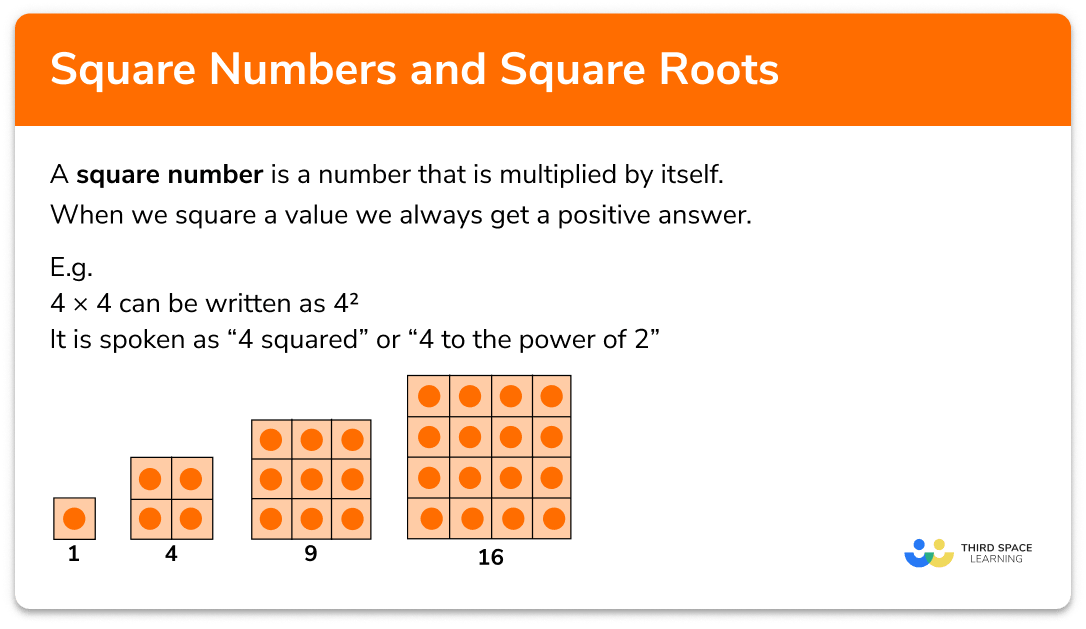Squares and square roots

Step by step guide, detailed examples, practice questions, exam questions and square numbers and square roots worksheets.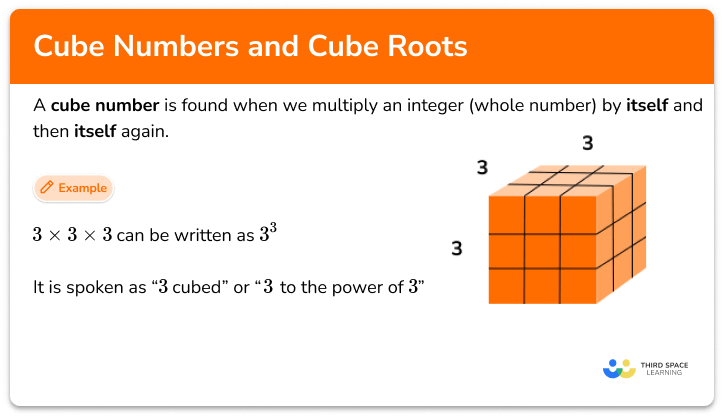Cubes and cube roots

Step by step guide, detailed examples, practice questions, exam questions and cube numbers and cube root worksheets.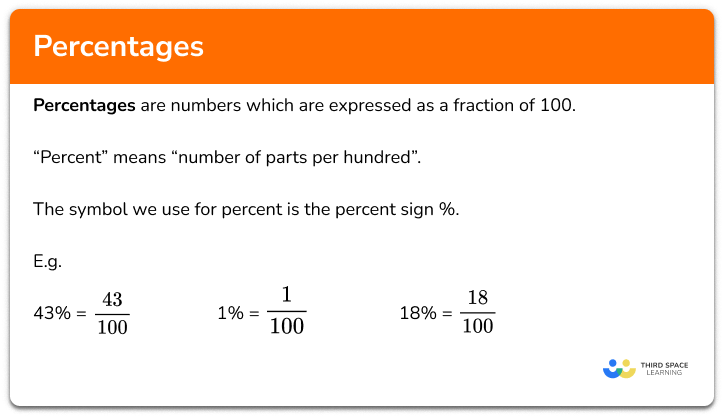Percentages

Step by step guide, detailed examples, practice questions, exam questions and how to work out percentage worksheets.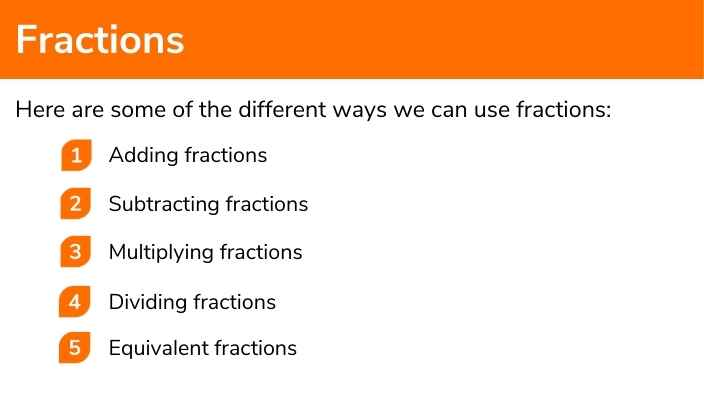Fractions

Step by step guide, detailed examples, practice questions, exam questions and fractions worksheets.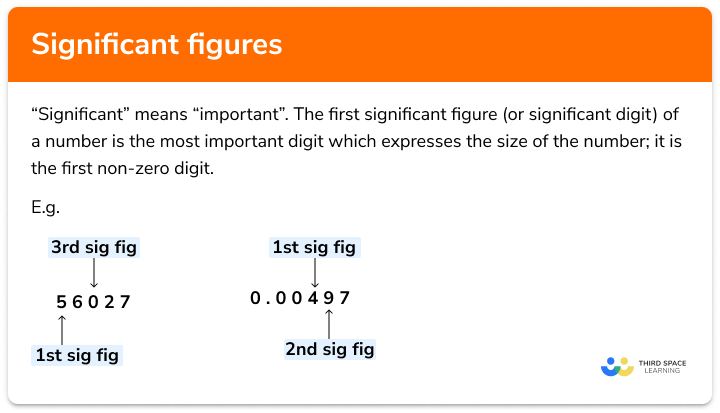Significant figures

Step by step guide, detailed examples, practice questions, exam questions and significant figures worksheets.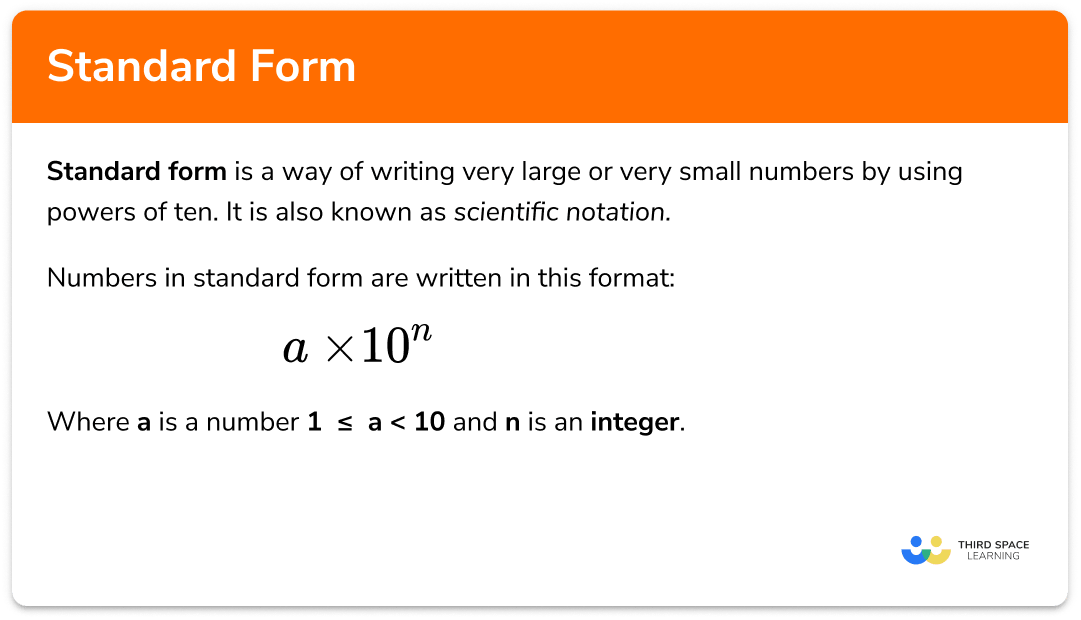Standard form

Step by step guide, detailed examples, practice questions, exam questions and standard form worksheets.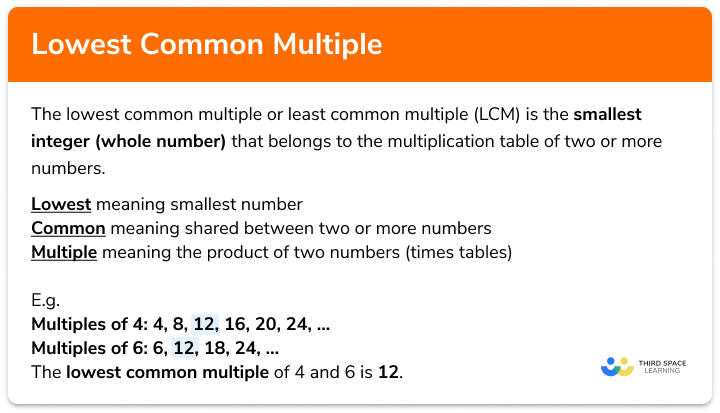Lowest common multiple

Step by step guide, detailed examples, practice questions, exam questions and lowest common multiple worksheets.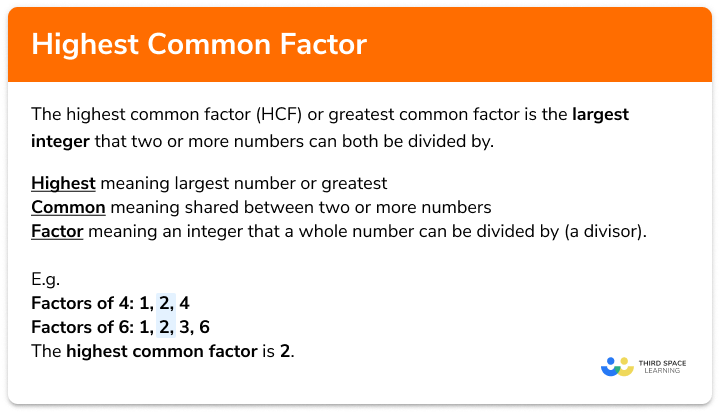Highest common factor

Step by step guide, detailed examples, practice questions, exam questions and Highest common factor worksheets.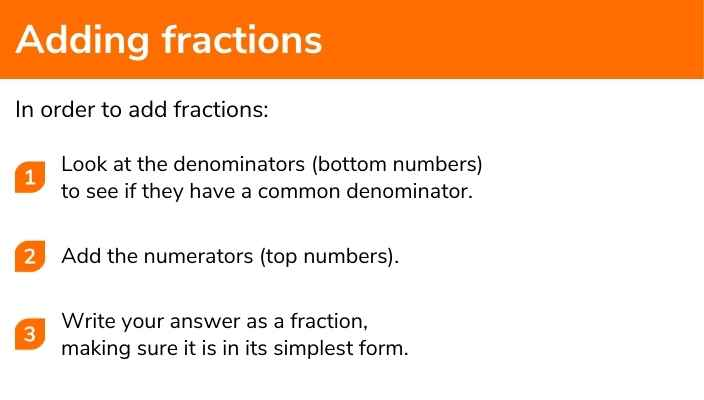Step by step guide, detailed examples, practice questions, exam questions and adding fractions worksheets.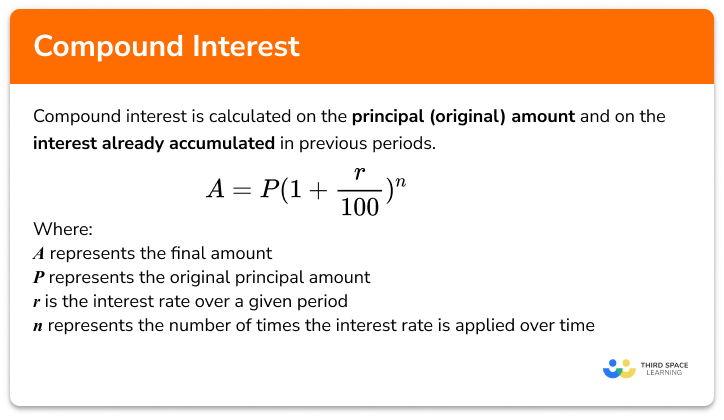Compound interest

Step by step guide, detailed examples, practice questions, exam questions and compound interest formula worksheets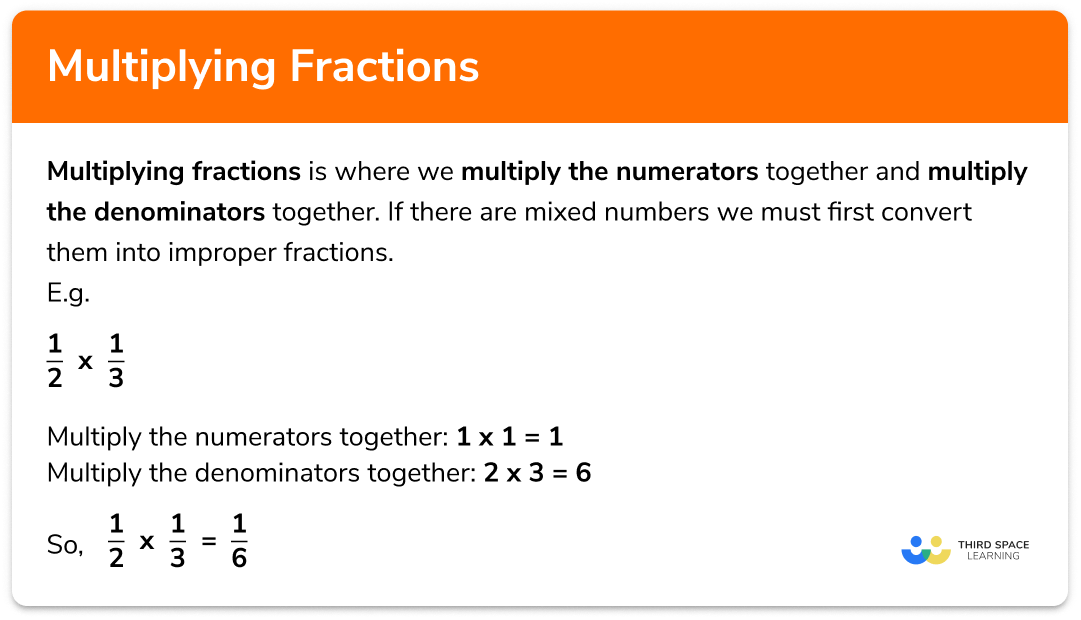Multiplying fractions

Step by step guide, detailed examples, practice questions, exam questions multiplying fractions worksheets.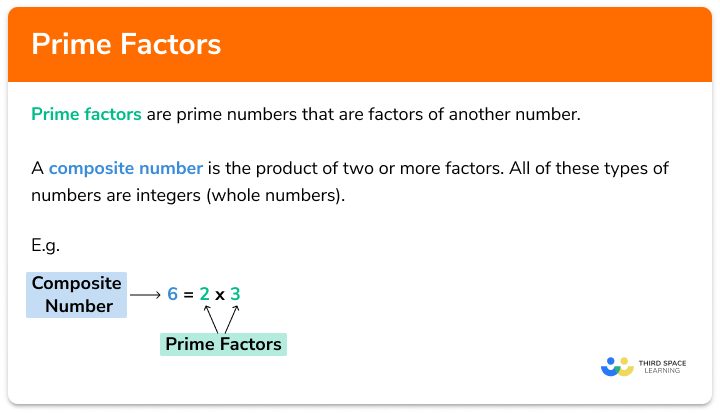Prime factors

Step by step guide, detailed examples, practice questions, exam questions and prime factors worksheets.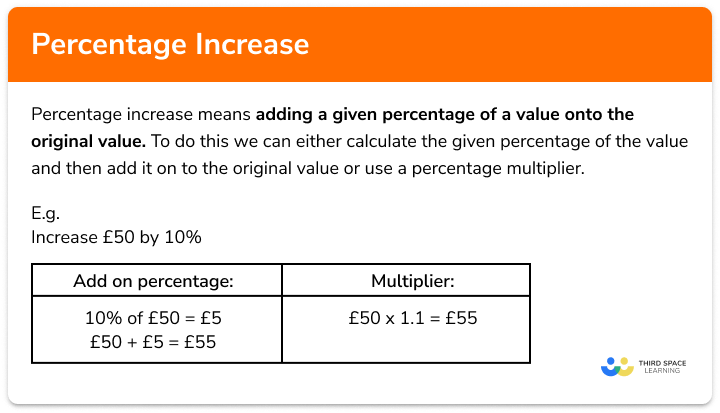Percentage increase

Step by step guide, detailed examples, practice questions, exam questions and how to work out percentage increase worksheets.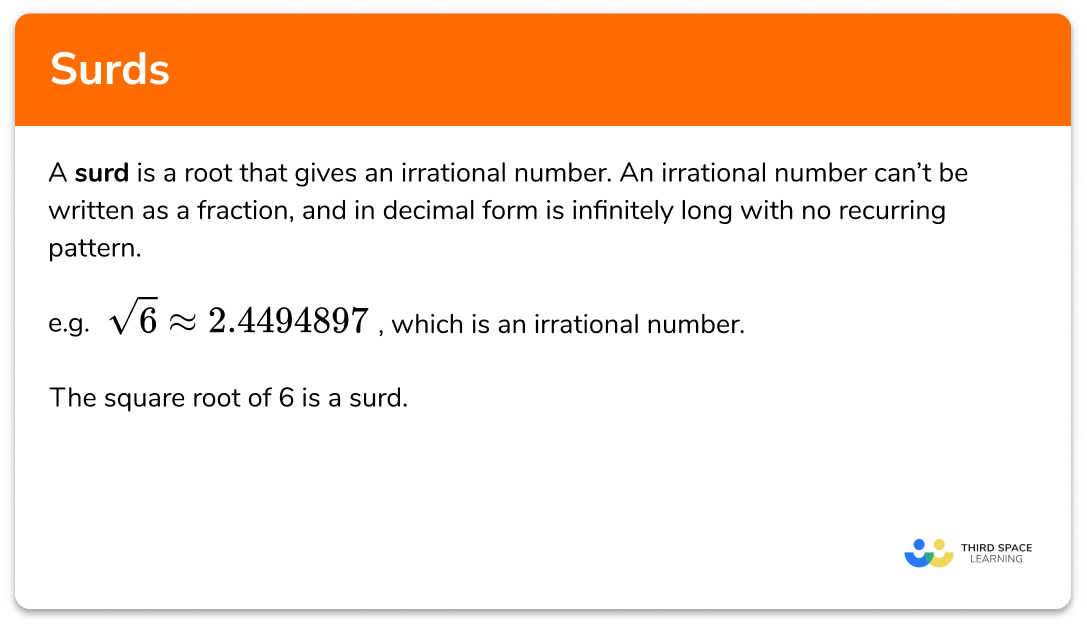Surds

Step by step guide, detailed examples, practice questions, exam questions and surds worksheets.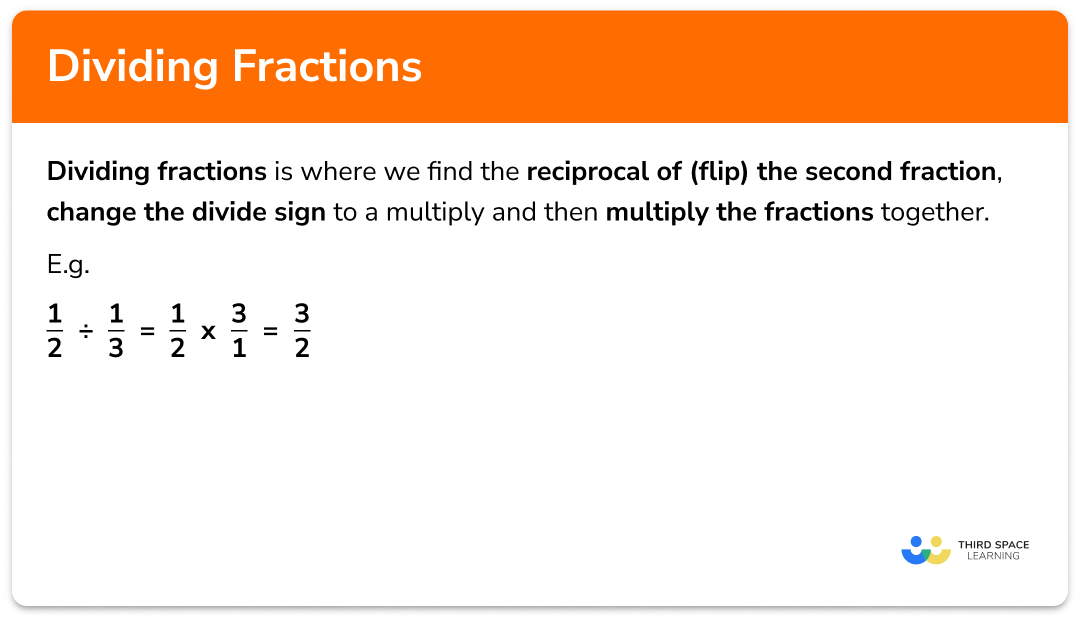Dividing fractions

Step by step guide, detailed examples, practice questions, exam questions and dividing fractions worksheets.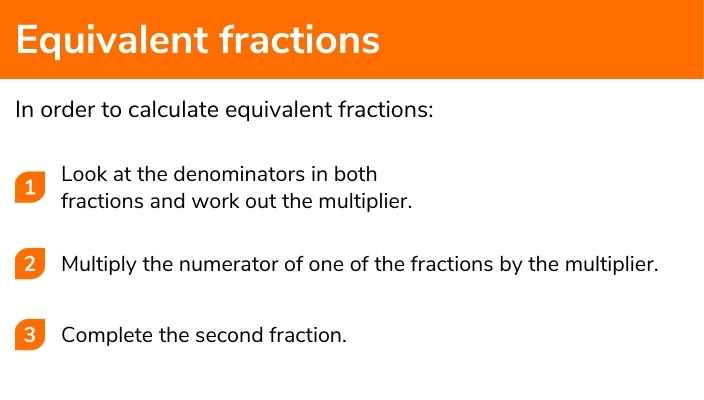Equivalent fractions

Step by step guide, detailed examples, practice questions, exam questions and equivalent fractions worksheets.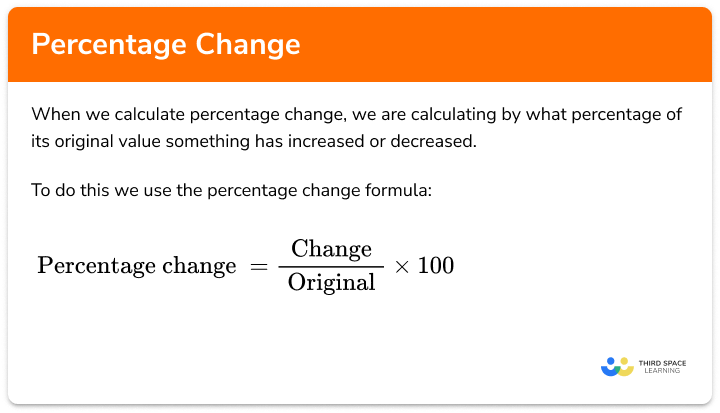Percentage change

Step by step guide, detailed examples, practice questions, exam questions and percentage change worksheets.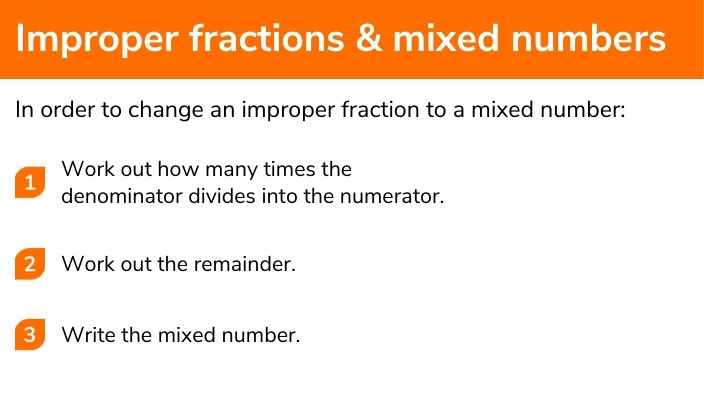Improper fraction to mixed number

Step by step guide, detailed examples, practice questions, exam questions, and improper fraction and mixed number worksheets.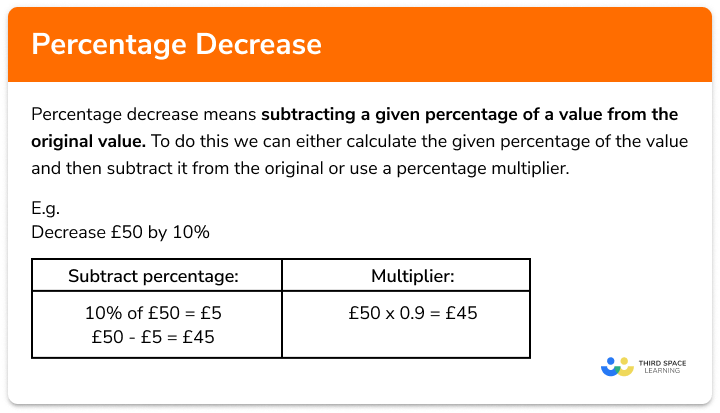Percentage decrease

Step by step guide, detailed examples, practice questions, exam questions and percentage decrease worksheets.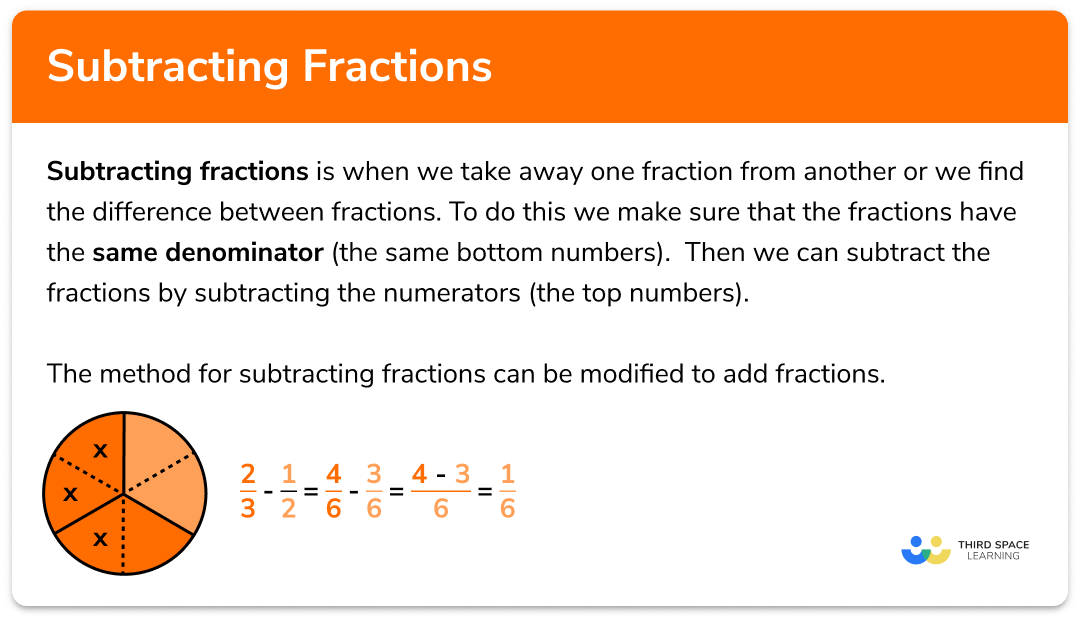Subtracting fractions

Step by step guide, detailed examples, practice questions, exam questions and subtracting fractions worksheets.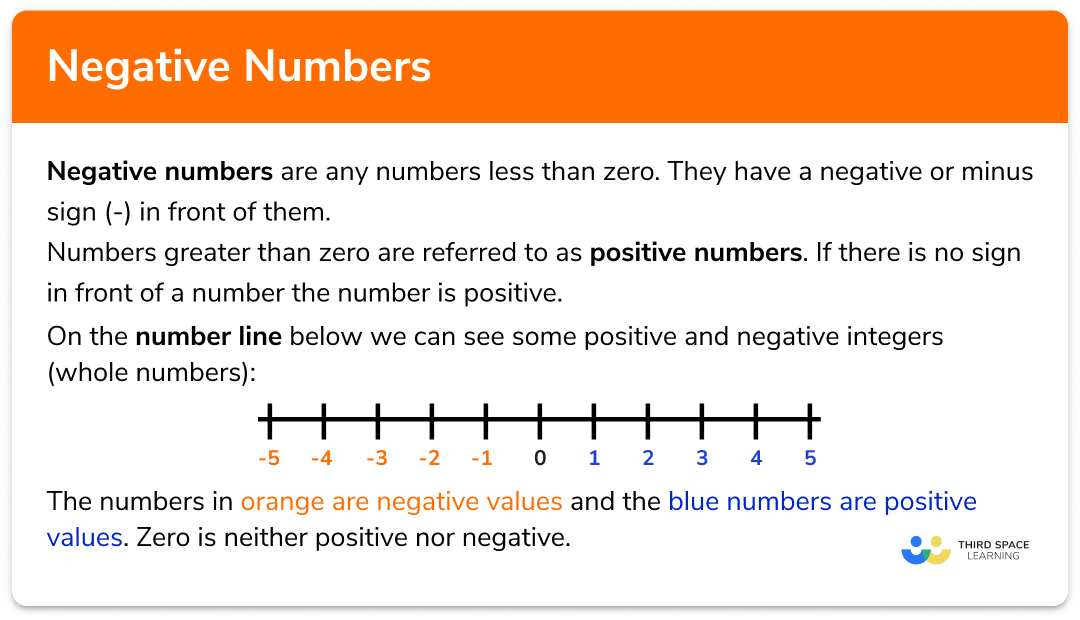Negative numbers

Step by step guide, detailed examples, practice questions, exam questions and negative numbers worksheets.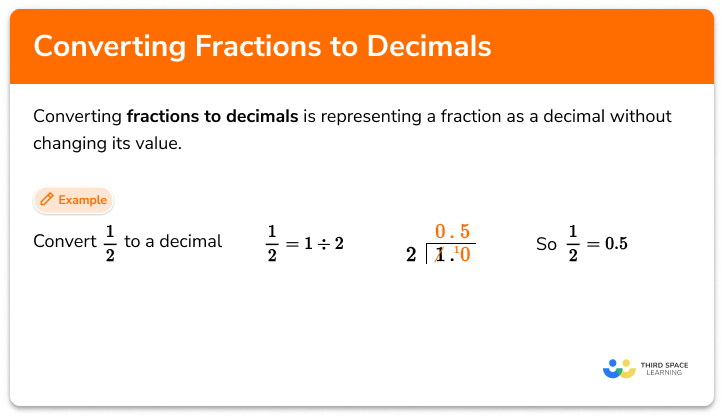Fractions to decimals

Step by step guide, detailed examples, practice questions, exam questions and fractions to decimals worksheets.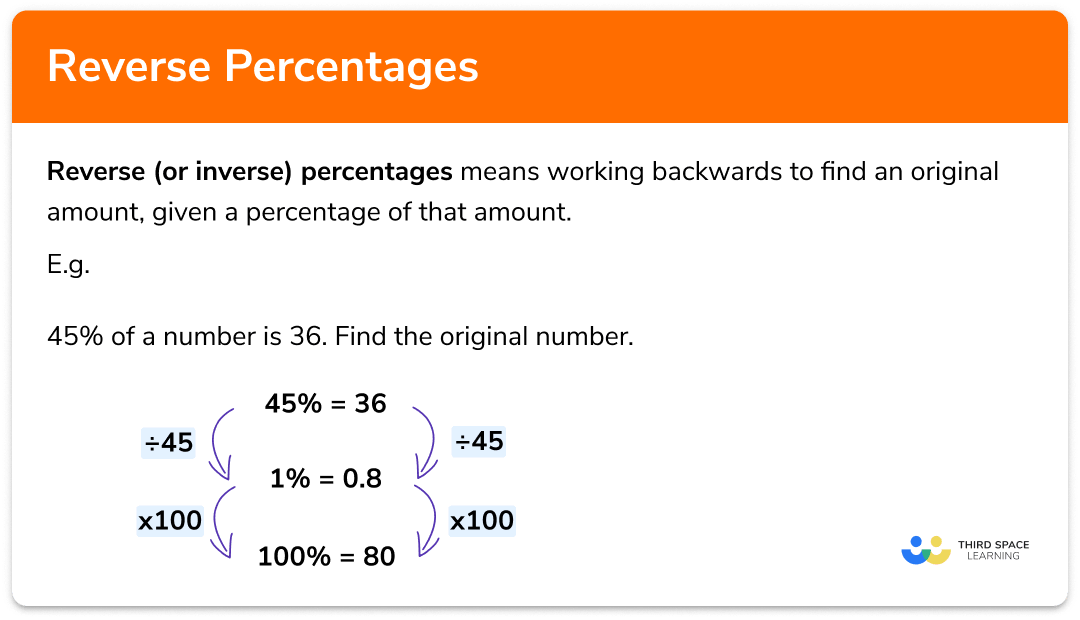Reverse percentages

Step by step guide, detailed examples, practice questions, exam questions and reverse percentages worksheets.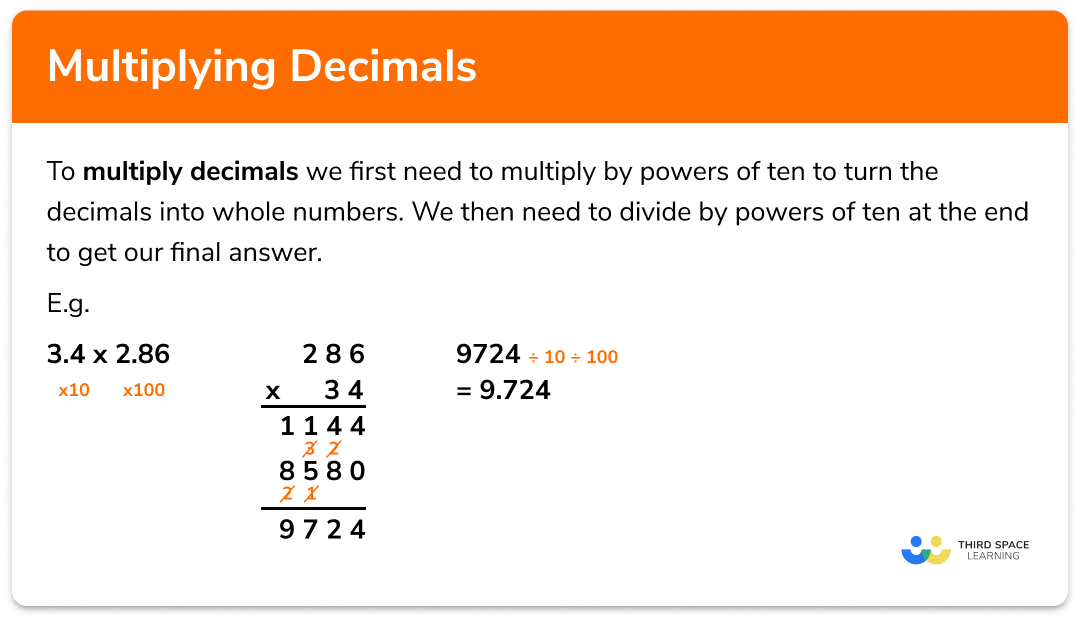Multiplying decimals

Step by step guide, detailed examples, practice questions, exam questions and multiplying decimals worksheets.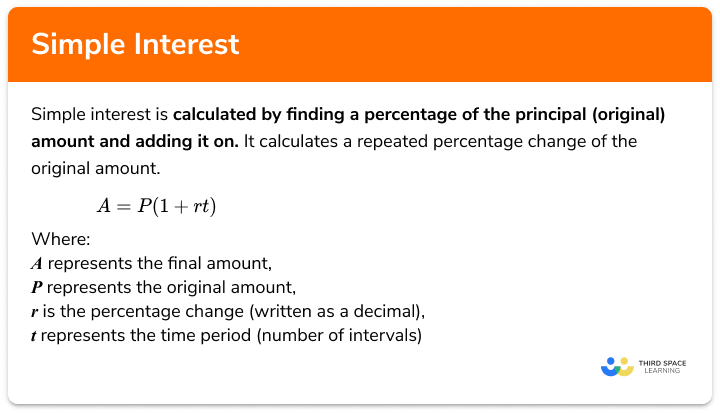Simple interest

Step by step guide, detailed examples, practice questions, exam questions and simple interest worksheets.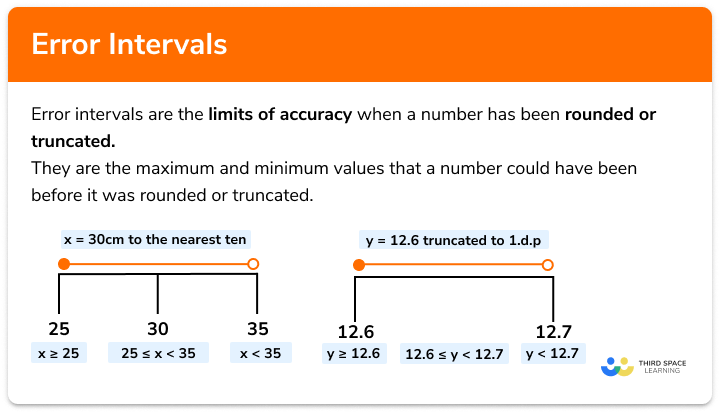Error intervals

Step by step guide, detailed examples, practice questions, exam questions and error intervals worksheets.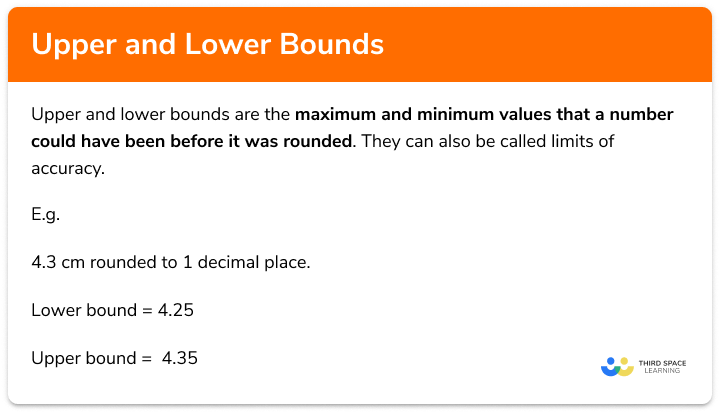Upper and lower bounds

Step by step guide, detailed examples, practice questions, exam questions and upper and lower bounds worksheets.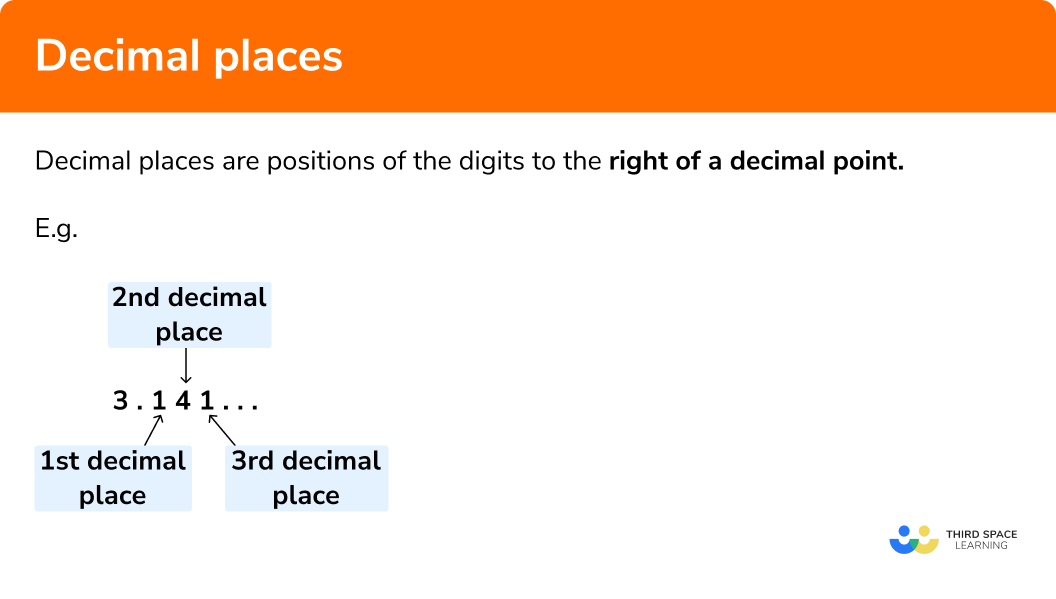Decimal places

Step by step guide, detailed examples, practice questions, exam questions and Decimal Places worksheets.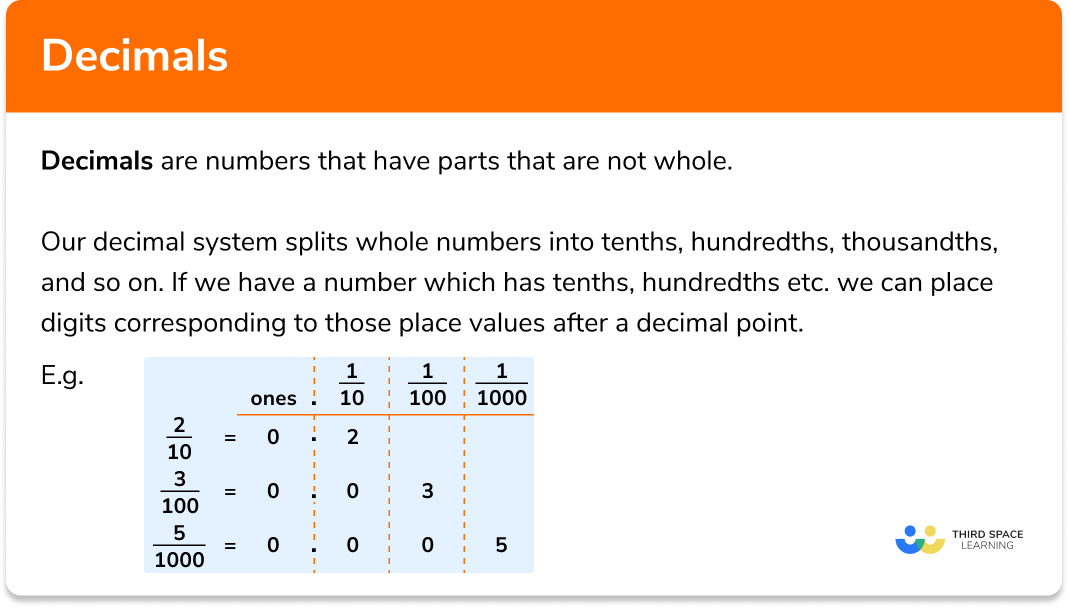Decimals

Step by step guide, detailed examples, practice questions, exam questions and decimals worksheets.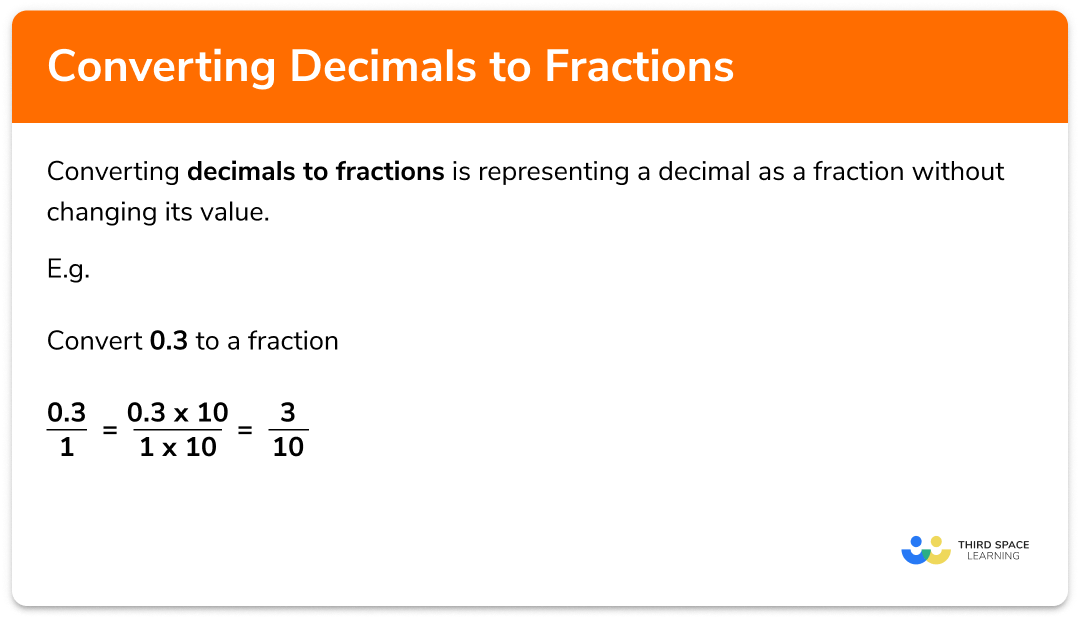Decimals to fractions

Step by step guide, detailed examples, practice questions, exam questions and converting decimals to fractions worksheets.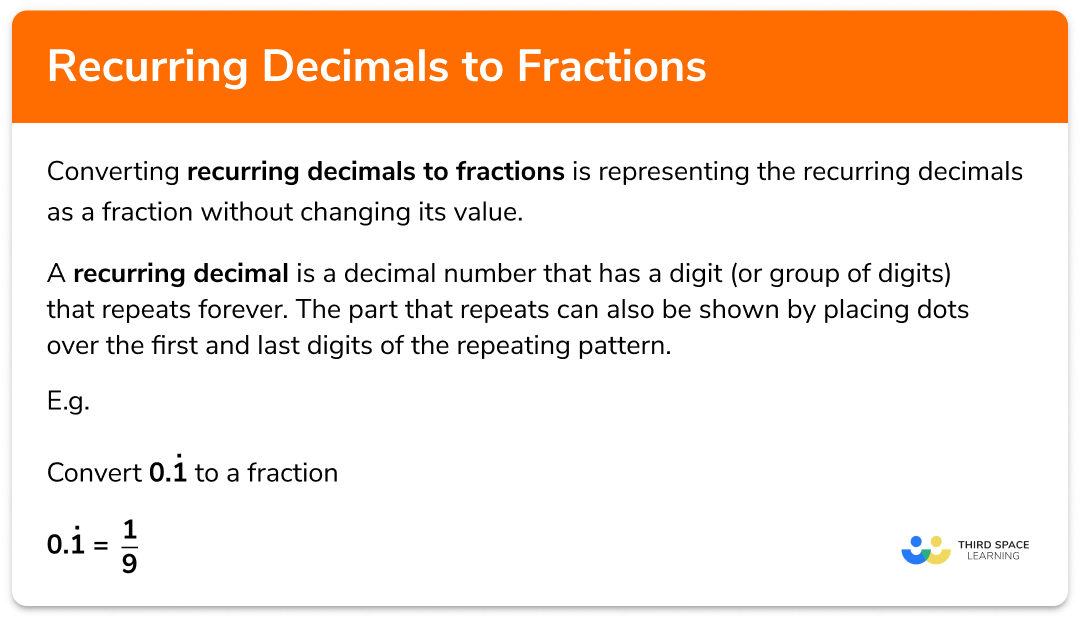Recurring decimals to fractions

Step by step guide, detailed examples, practice questions, exam questions and recurring decimals to fractions worksheets.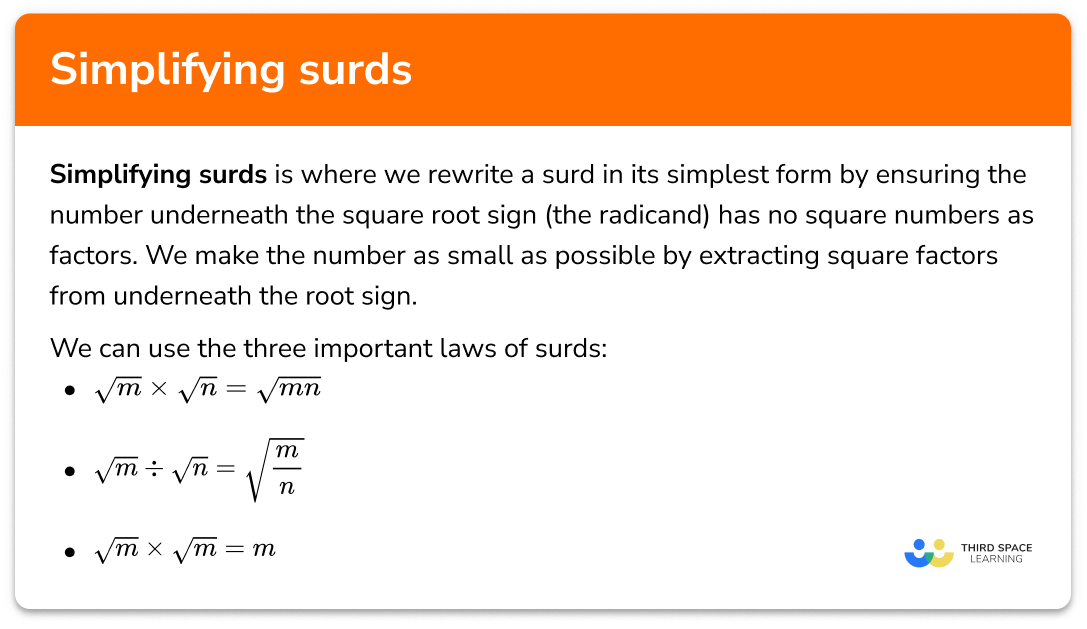Simplifying surds

Step by step guide, detailed examples, practice questions, exam questions and simplifying surds worksheets.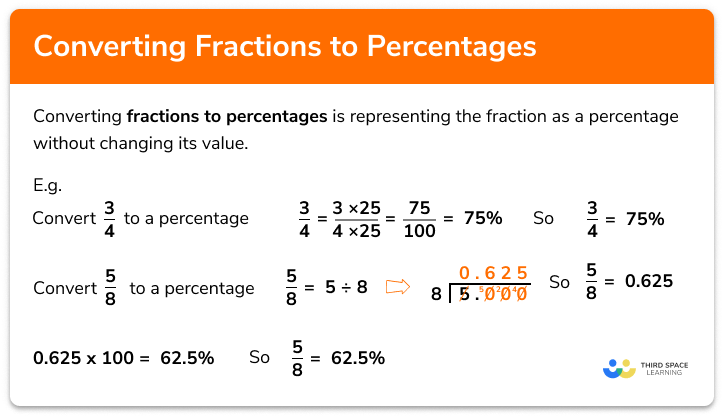Fractions to percentages

Step by step guide, detailed examples, practice questions, exam questions and fractions to percentages worksheets.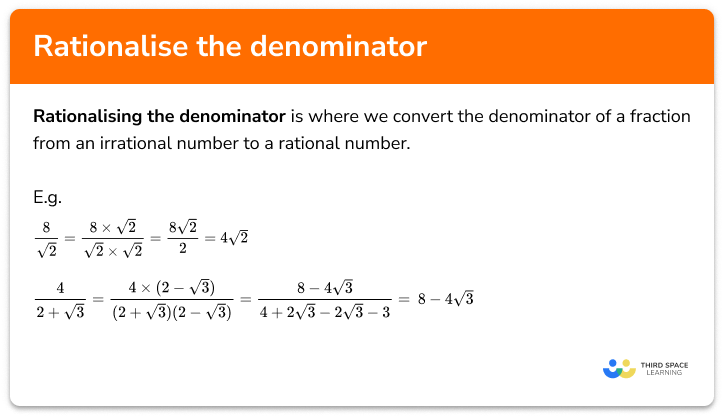Rationalise the denominator

Step by step guide, detailed examples, practice questions, exam questions rationalise the denominator worksheets.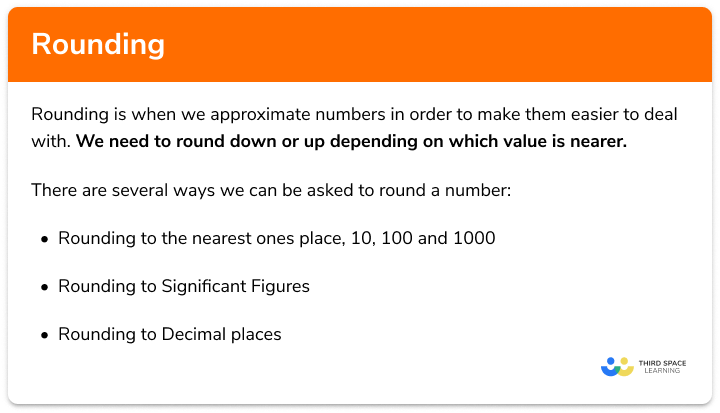Rounding numbers

Step by step guide, detailed examples, practice questions, exam questions and rounding numbers worksheets.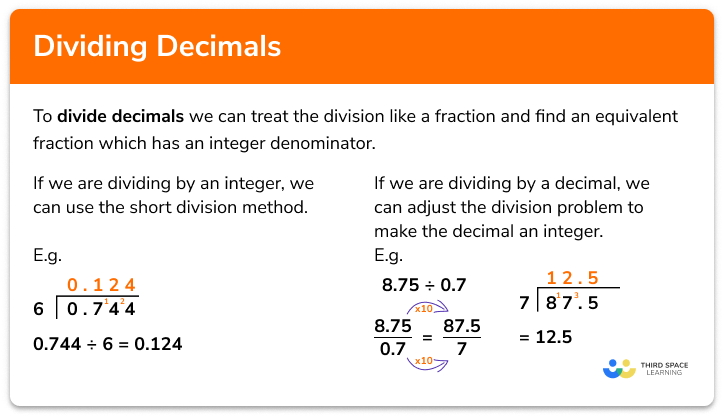Dividing decimals

Step by step guide, detailed examples, practice questions, exam questions dividing decimals worksheets.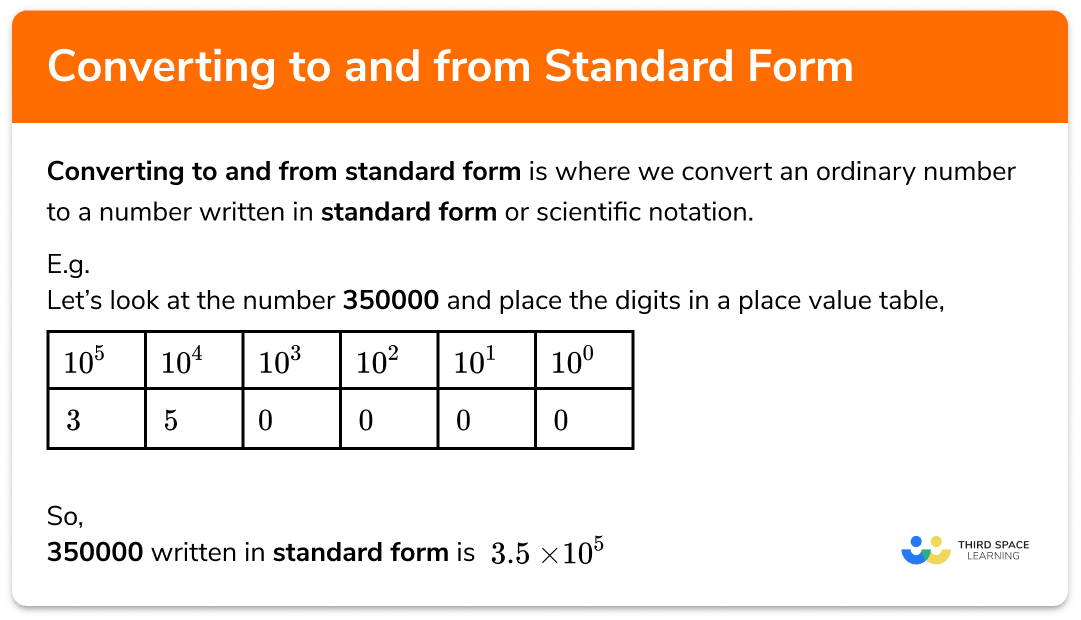Converting to and from standard form

Step by step guide, detailed examples, practice questions, exam questions and standard form calculator worksheets.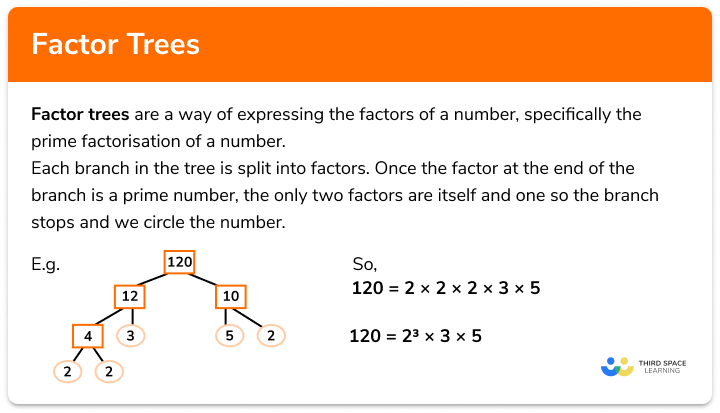Factor trees

Step by step guide, detailed examples, practice questions, exam questions and factor tree worksheets.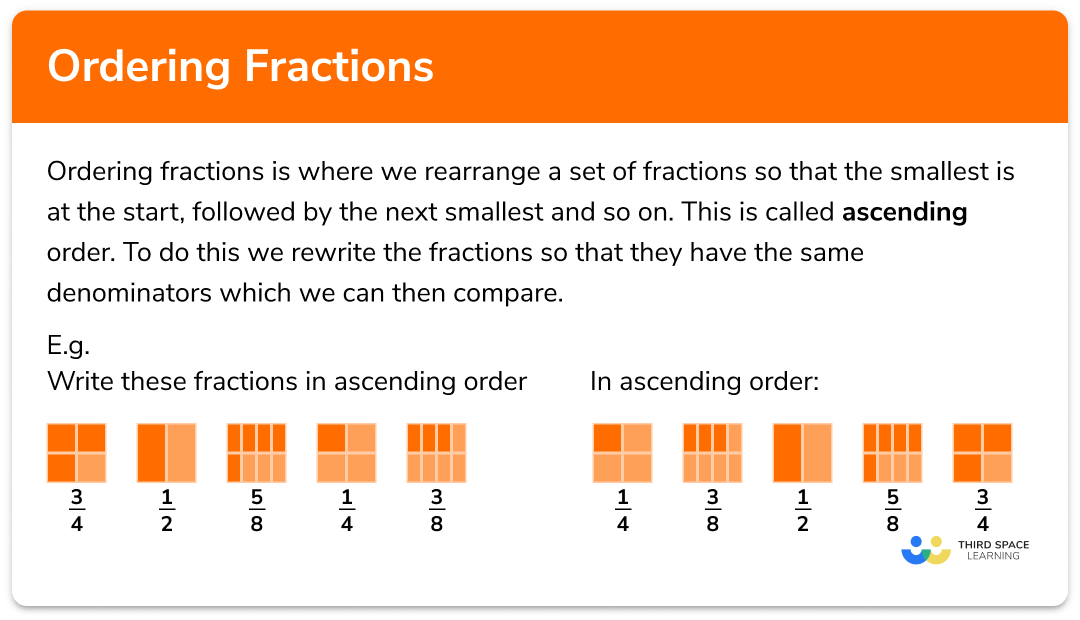Ordering fractions

Step by step guide, detailed examples, practice questions, exam questions and ordering fractions worksheets.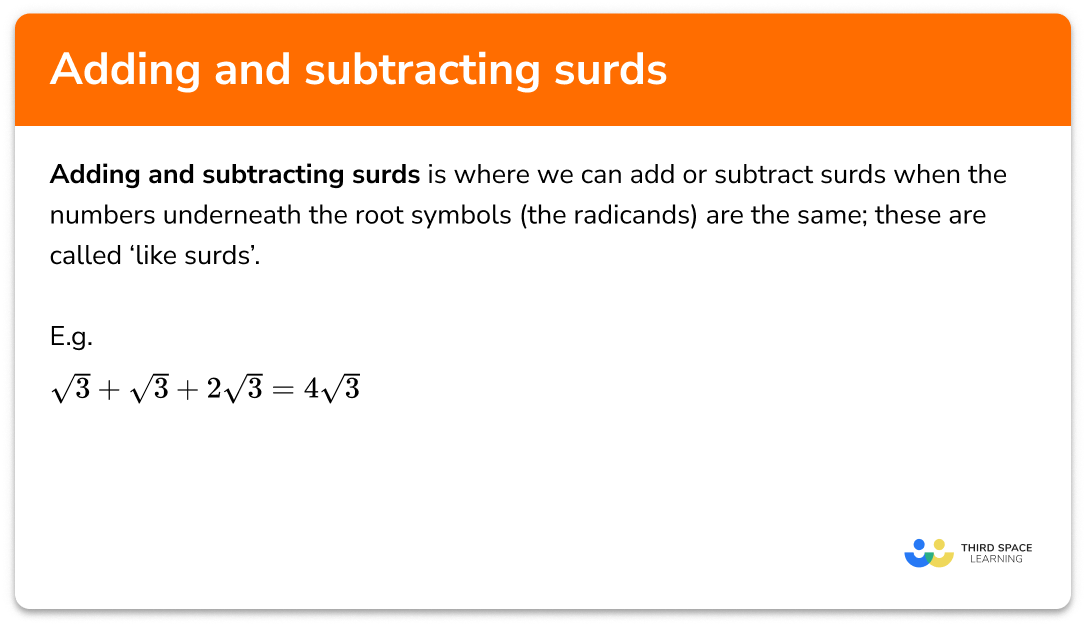Step by step guide, detailed examples, practice questions, exam questions and adding and adding and subtracting surds worksheets.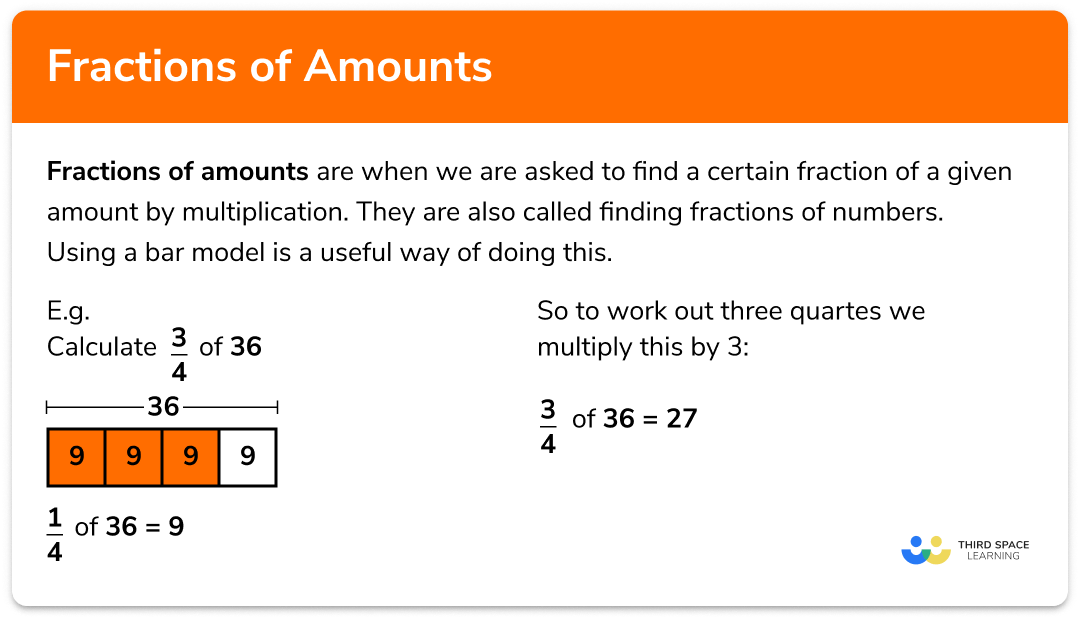Fractions of amounts

Step by step guide, detailed examples, practice questions, exam questions and fractions of amounts worksheets.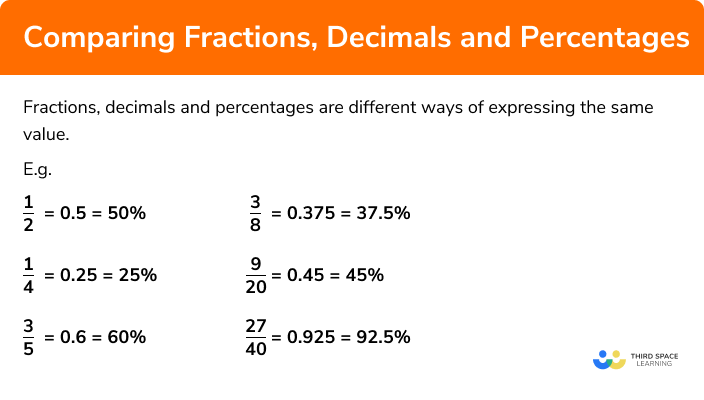Converting fractions, decimals and percentages

Step by step guide, detailed examples, practice questions, exam questions comparing fractions, decimals and percentages worksheets.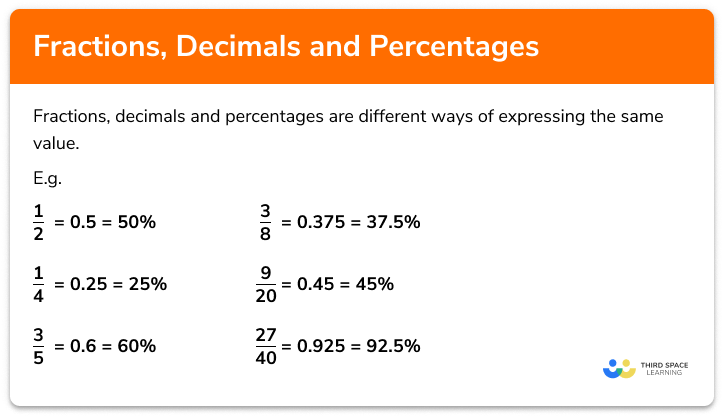Fractions, decimals and percentages

Step by step guide, detailed examples, practice questions, exam questions and fractions, decimals and percentages worksheets.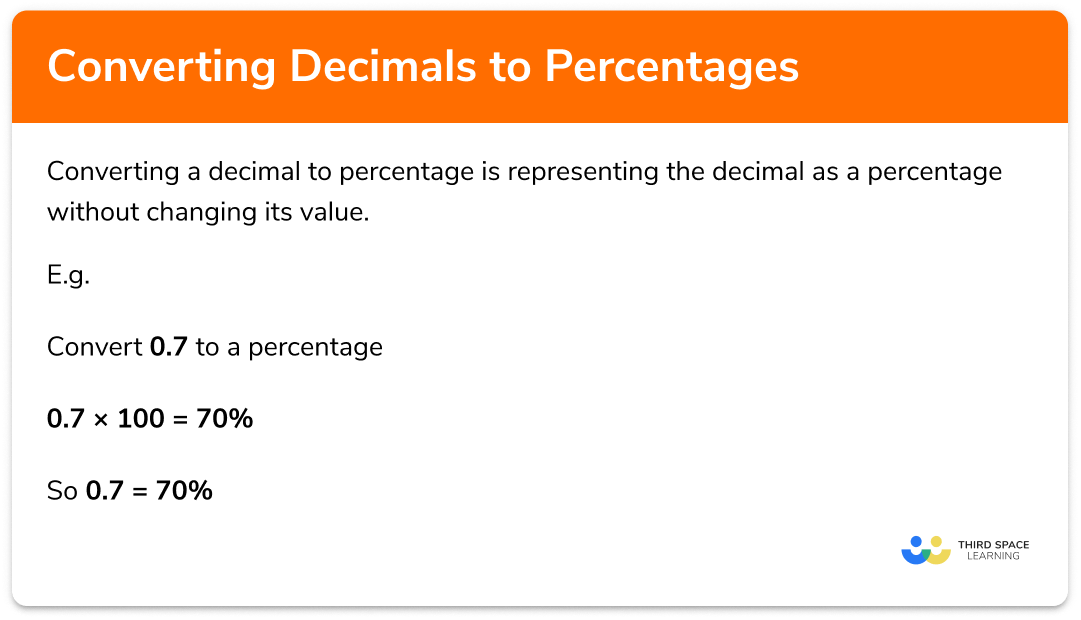Decimal to percentage

Step by step guide, detailed examples, practice questions, exam questions and decimal to percentage worksheets.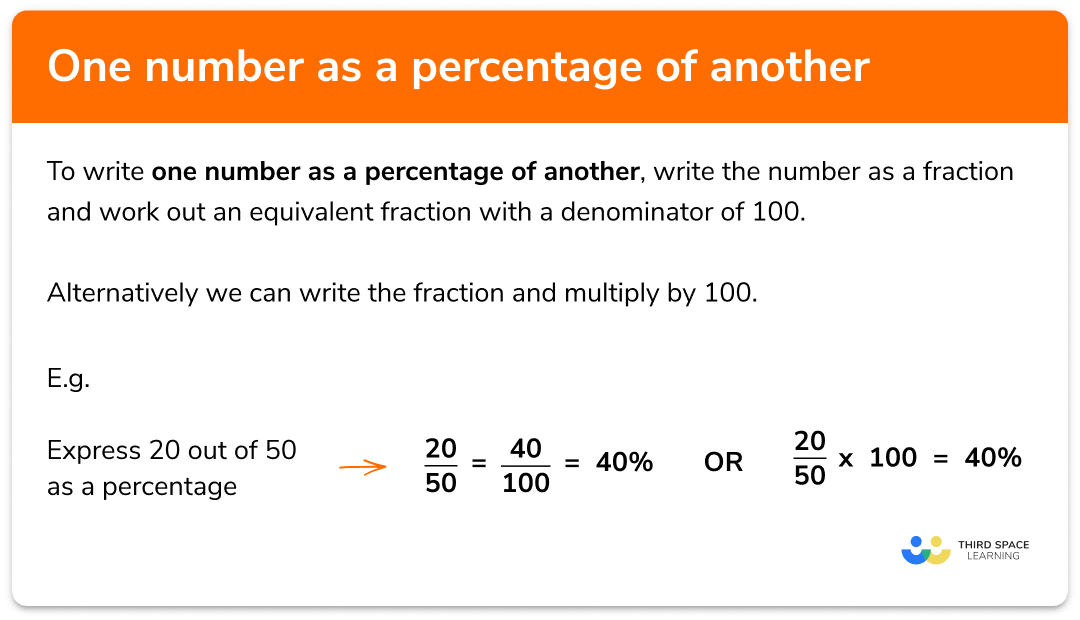One number as a percentage of another

Step by step guide, detailed examples, practice questions, exam questions and one number as a percentage of another worksheets.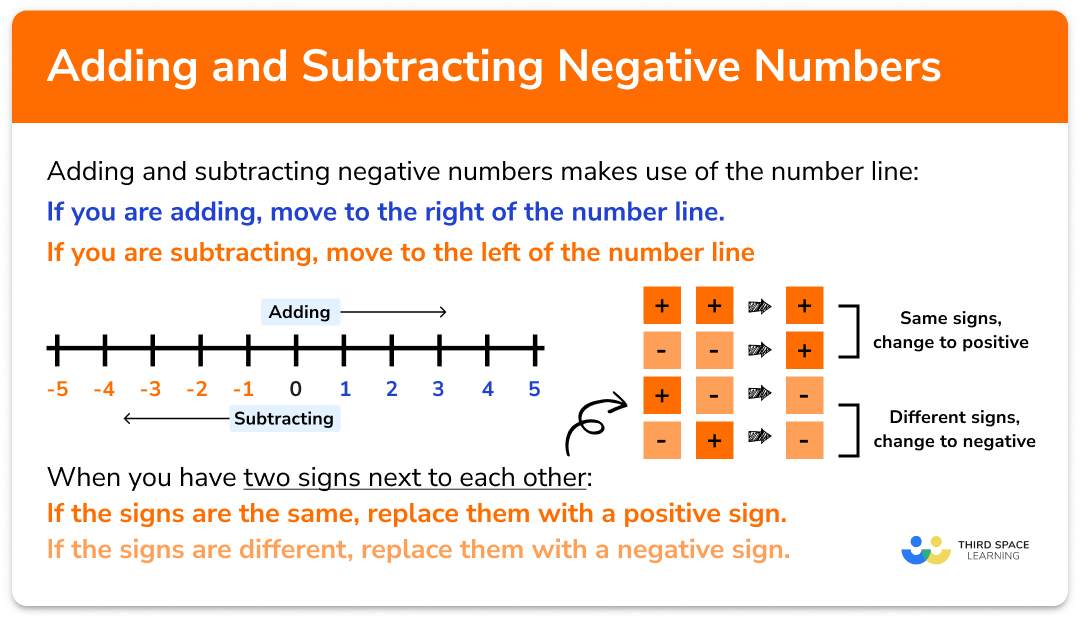Step by step guide, detailed examples, practice questions, exam questions and adding and subtracting negative numbers worksheets.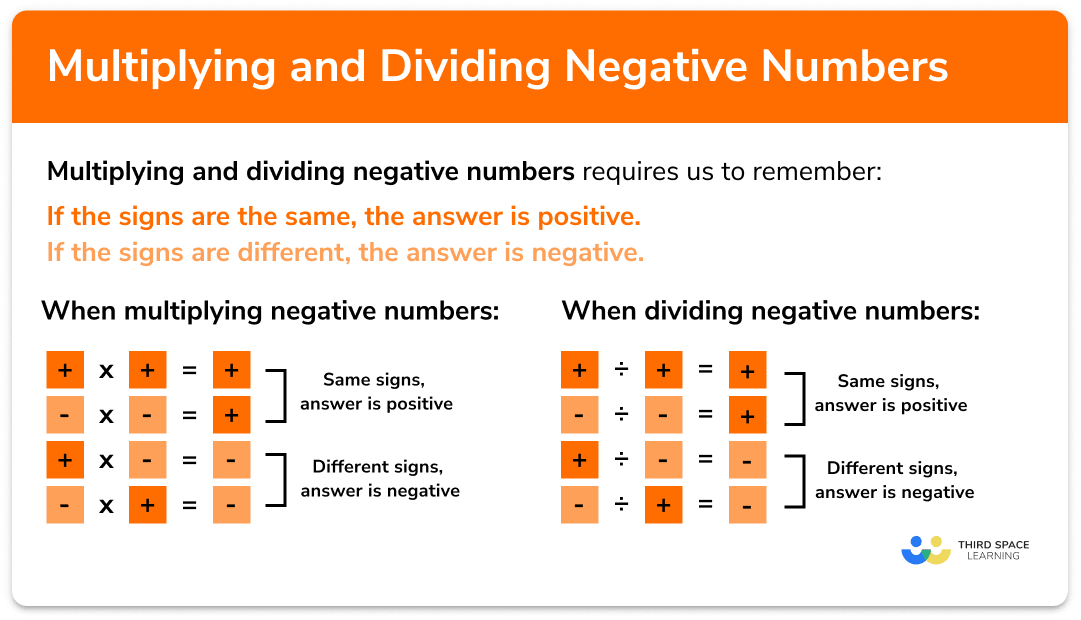Multiplying and dividing negative numbers

Step by step guide, detailed examples, practice questions, exam questions and multiplying and dividing negative numbers worksheets.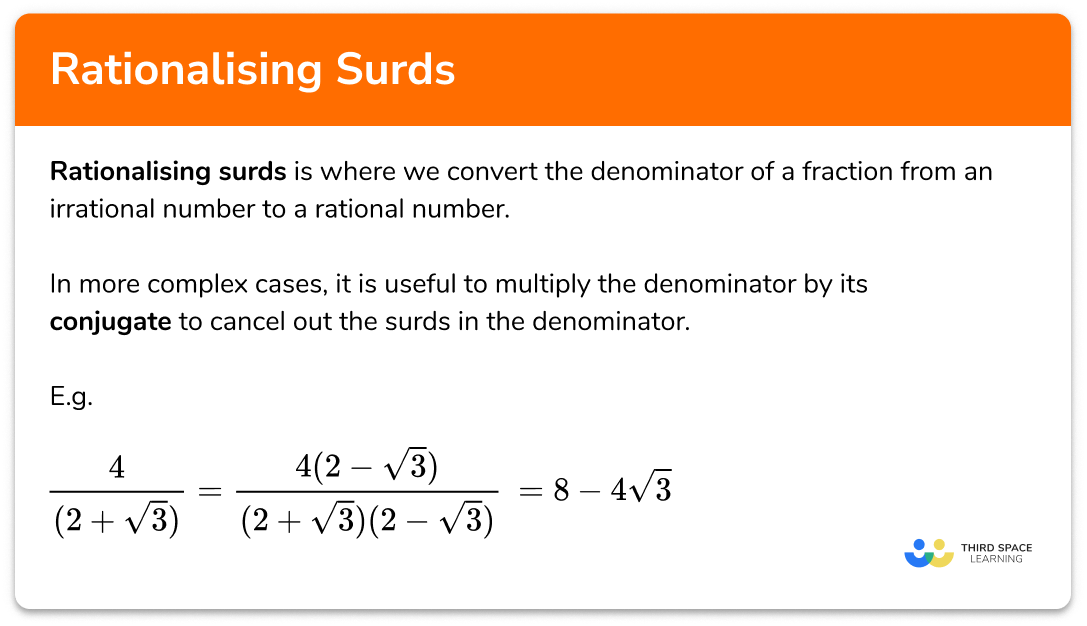Rationalising surds

Step by step guide, detailed examples, practice questions, exam questions and rationalising surds worksheets.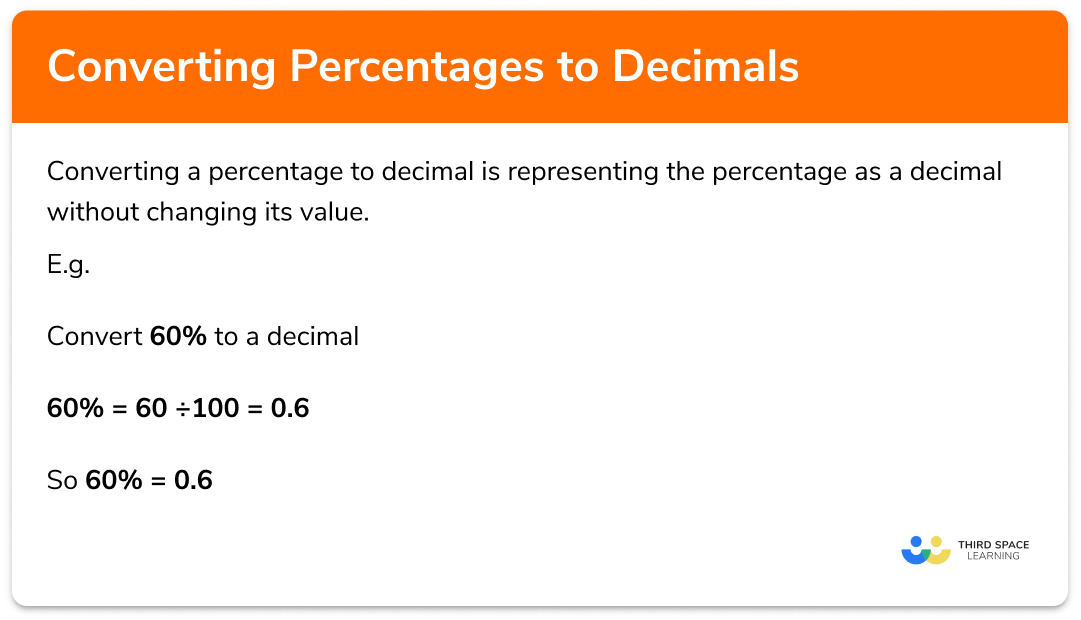Percentage to decimal

Step by step guide, detailed examples, practice questions, exam questions and percentage to decimal worksheets.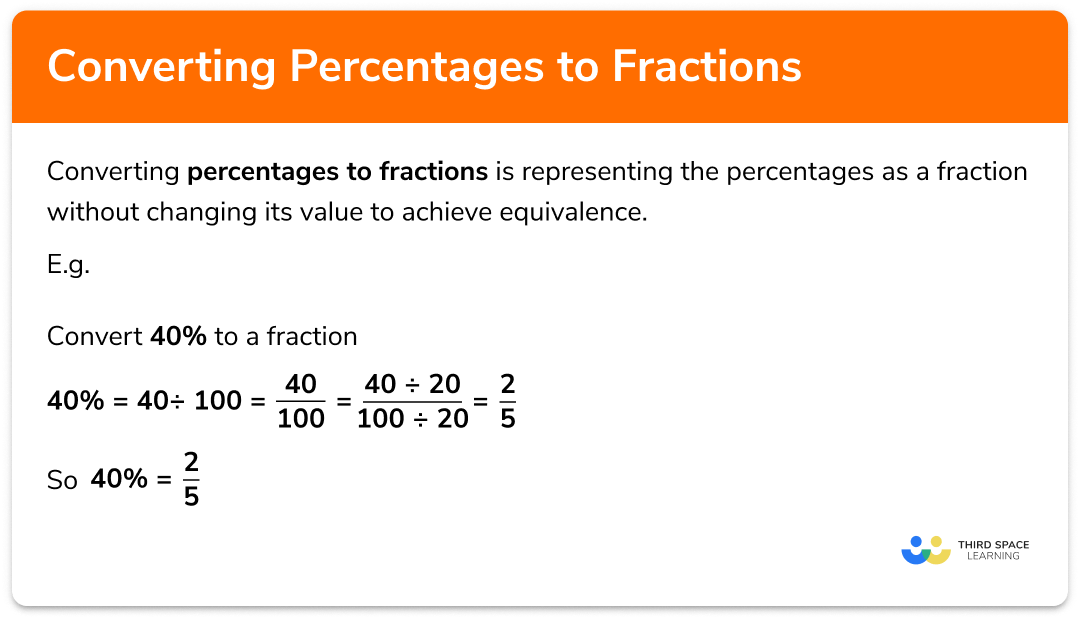Percentages to fractions

Step by step guide, detailed examples, practice questions, exam questions and percentages to fractions worksheets.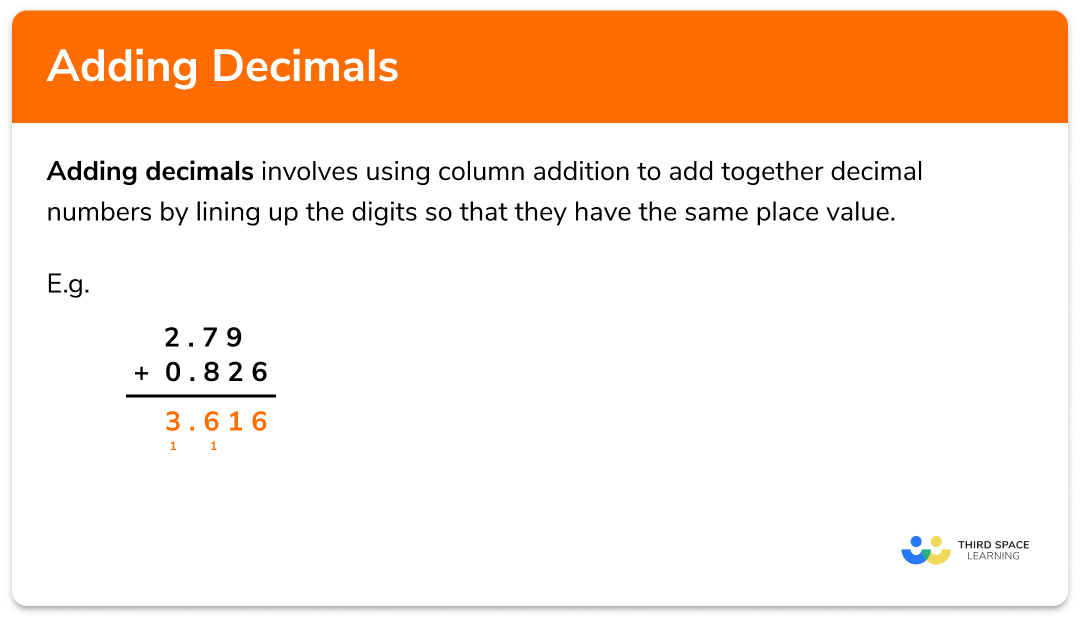Step by step guide, detailed examples, practice questions, exam questions and adding decimals worksheets.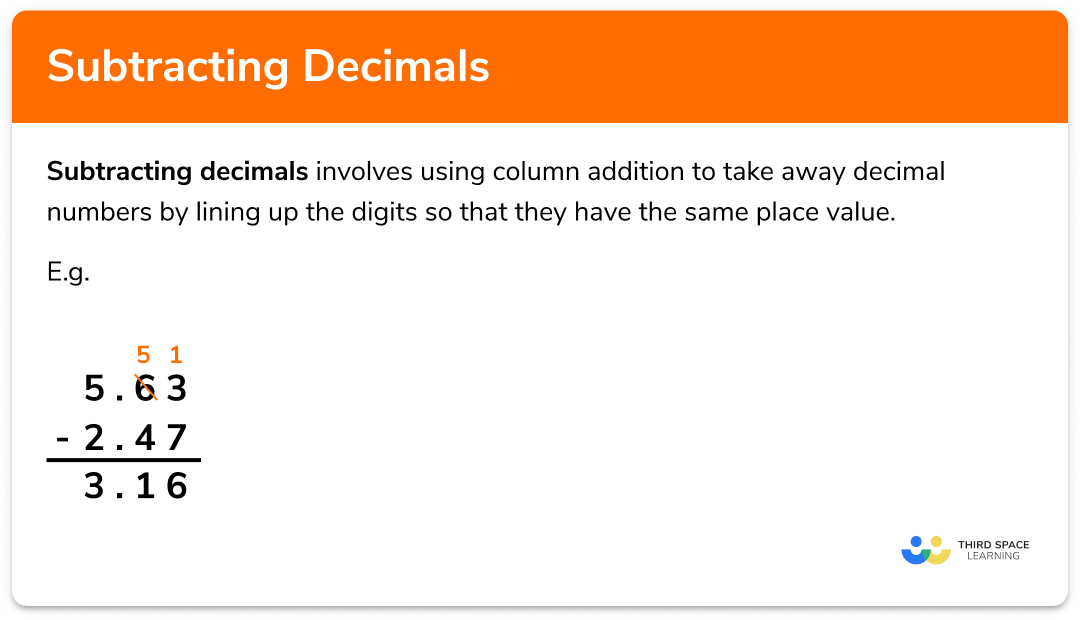Subtracting decimals

Step by step guide, detailed examples, practice questions, exam questions and subtracting decimals worksheets.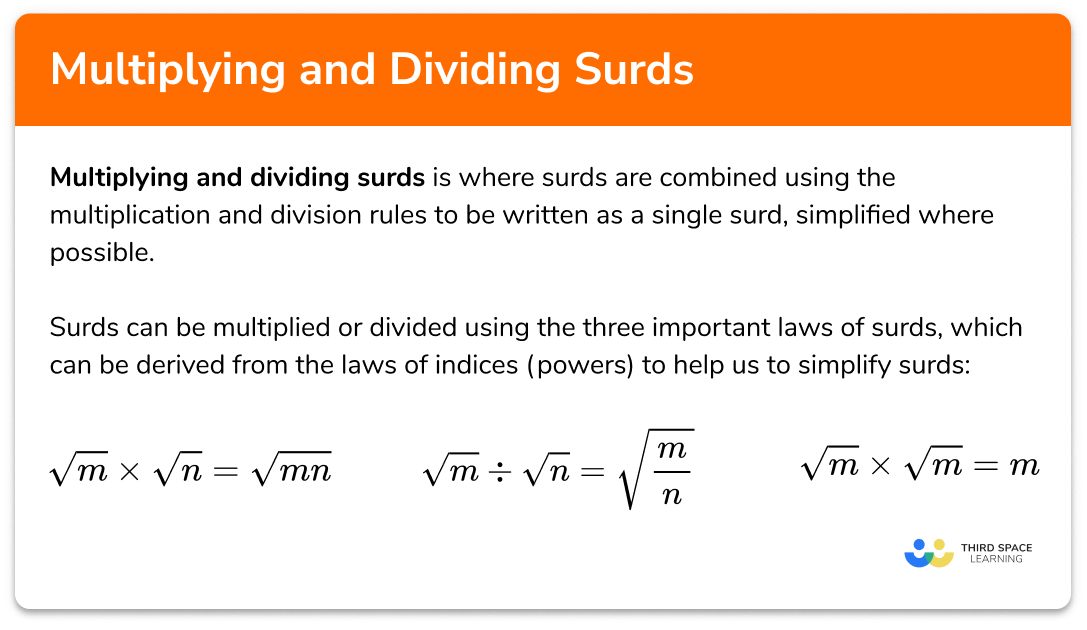Multiplying and dividing surds

Step by step guide, detailed examples, practice questions, exam questions and multiplying and dividing surds worksheets.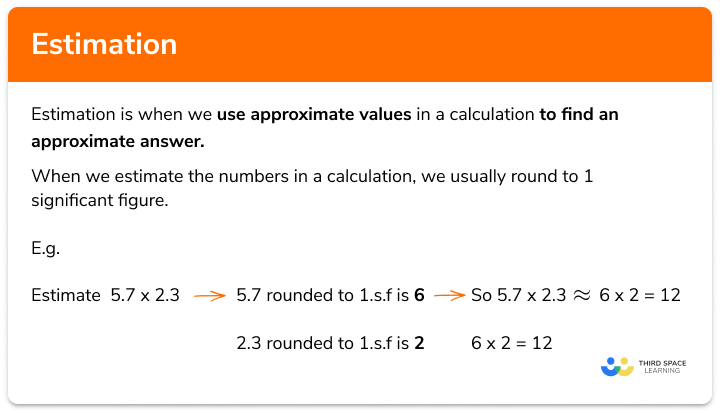Estimation

Step by step guide, detailed examples, practice questions, exam questions and estimation worksheets.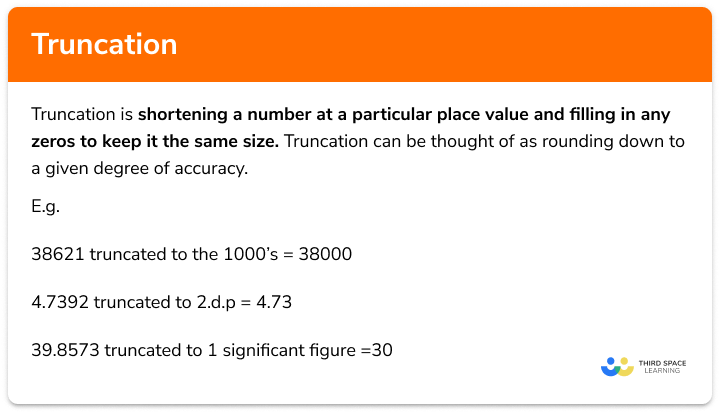Truncation

Step by step guide, detailed examples, practice questions, exam questions and truncation worksheets.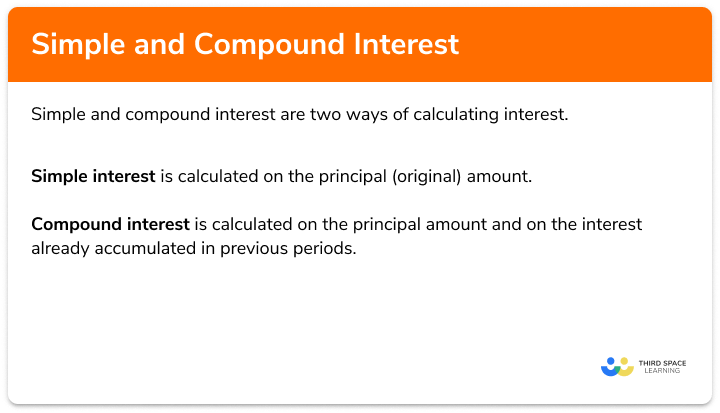Simple interest and compound interest

Step by step guide, detailed examples, practice questions, exam questions and simple interest and compound interest worksheets.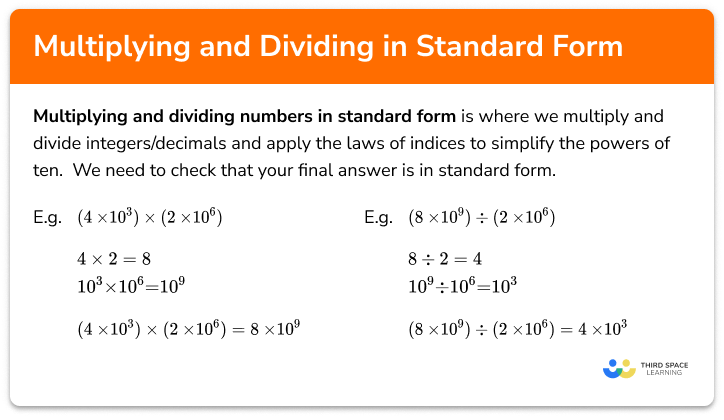Multiplying and dividing in standard form

Step by step guide, detailed examples, practice questions, exam questions and dividing standard form worksheets.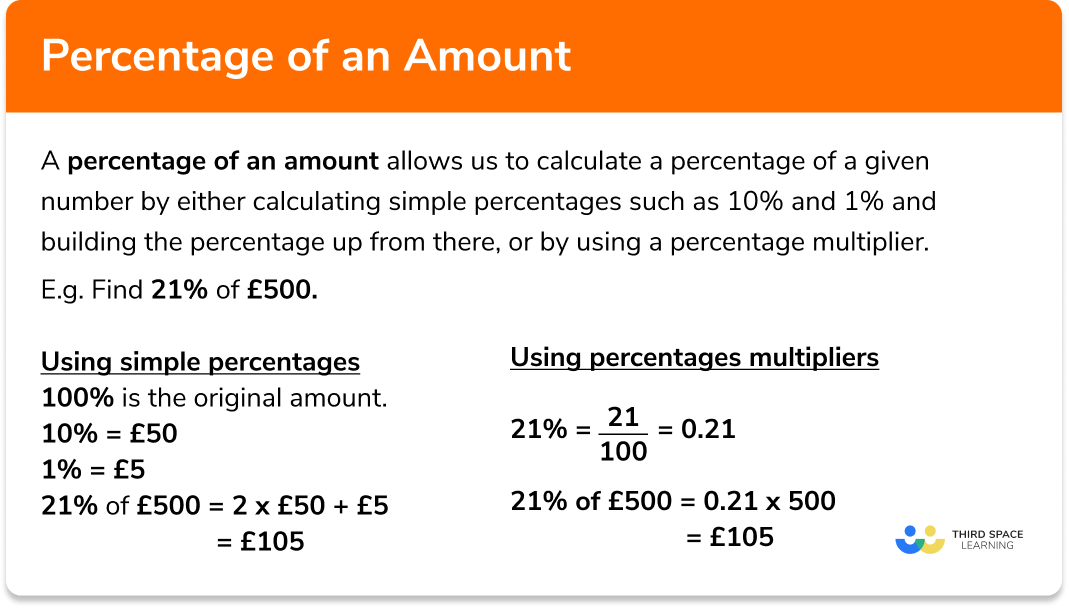Percentage of an amount

Step by step guide, detailed examples, practice questions, exam questions and percentage of an amount worksheets.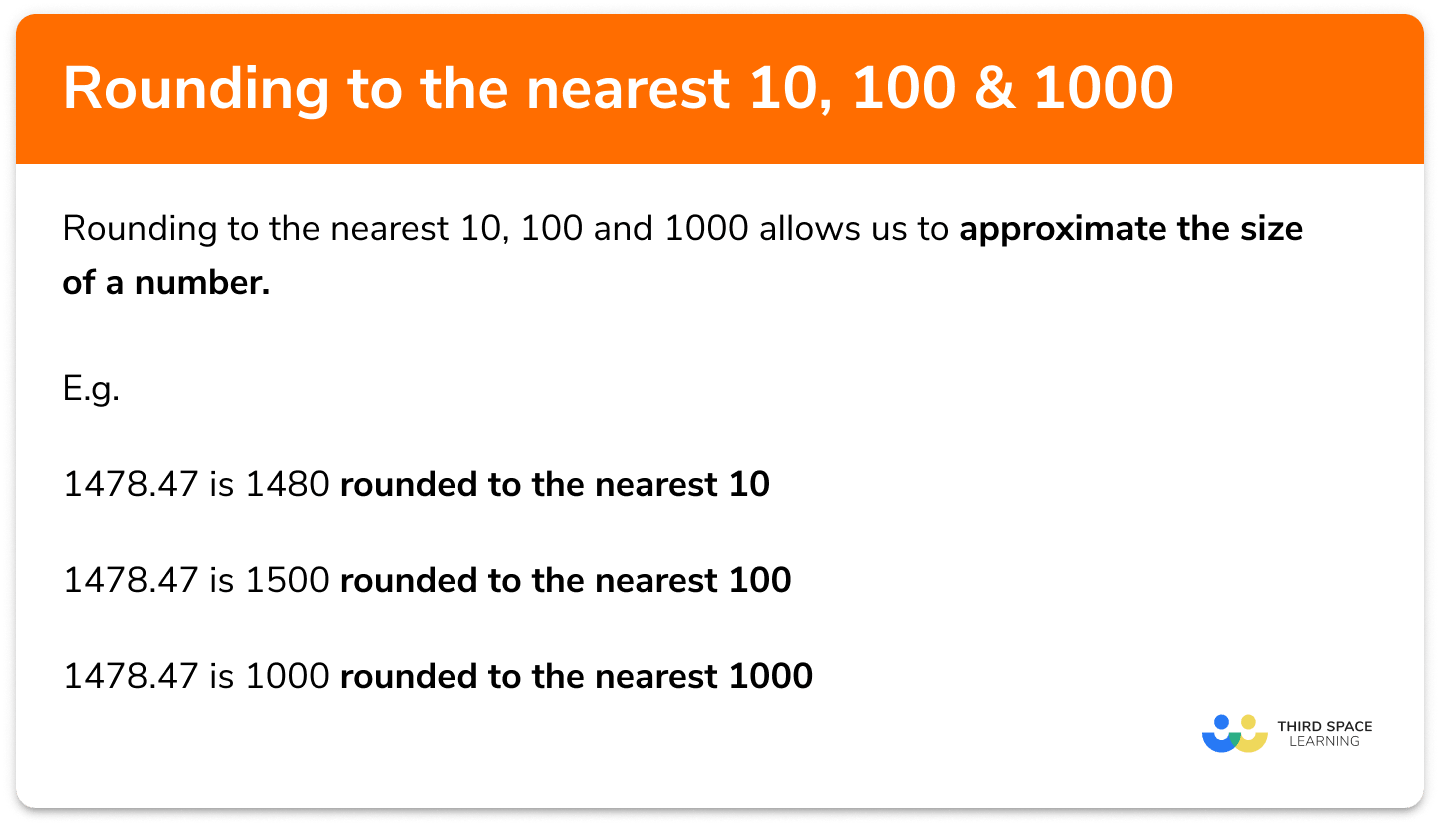Rounding to the nearest 10, 100, and 1000

Step by step guide, detailed examples, practice questions, exam questions and Rounding to the nearest 10 100 and 1000 worksheets.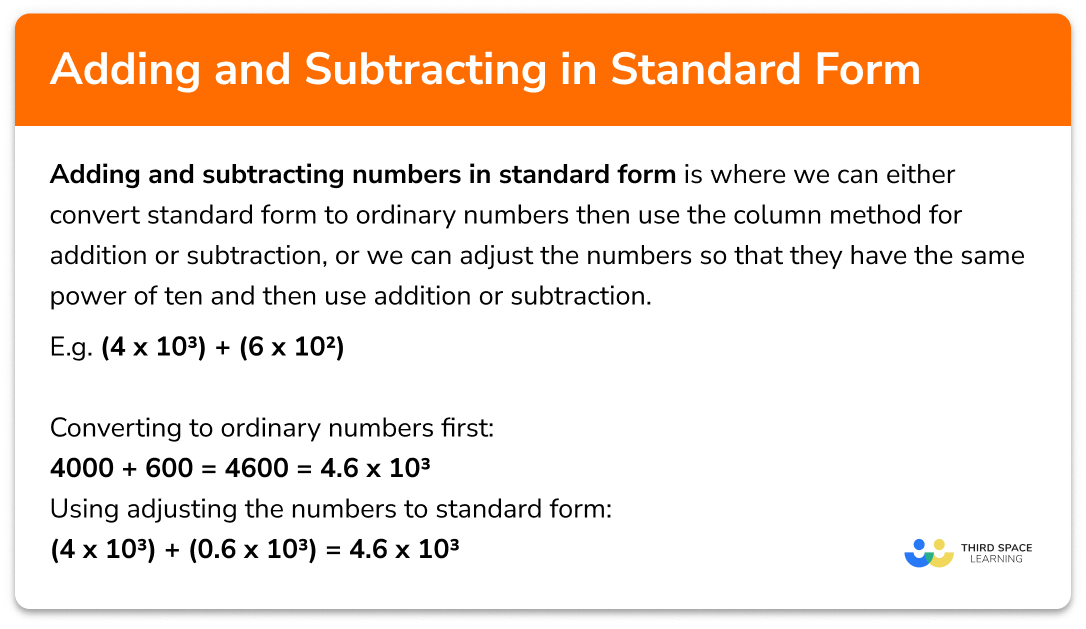Adding and subtracting in standard form

Step by step guide, detailed examples, practice questions, exam questions and adding standard form worksheets.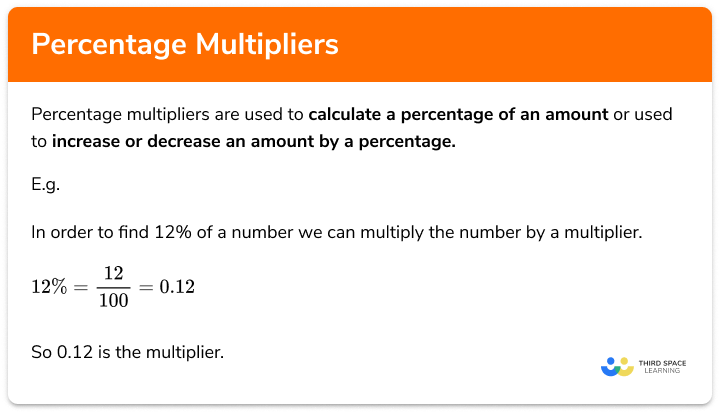Percentage multipliers

Step by step guide, detailed examples, practice questions, exam questions and percentage multiplier worksheets.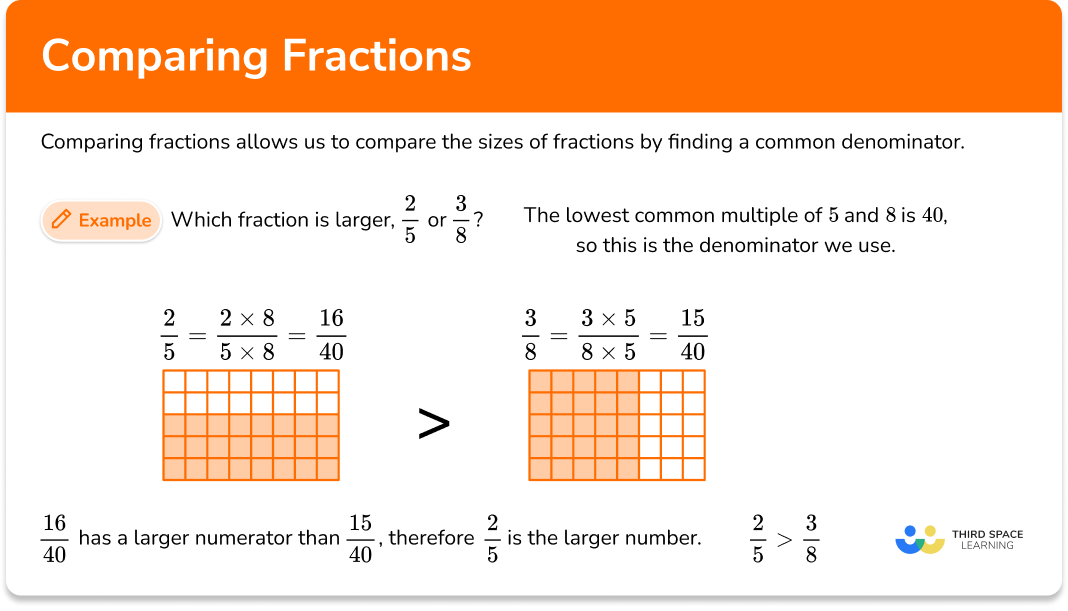Comparing fractions

Step by step guide, detailed examples, practice questions, exam questions and comparing fractions worksheets.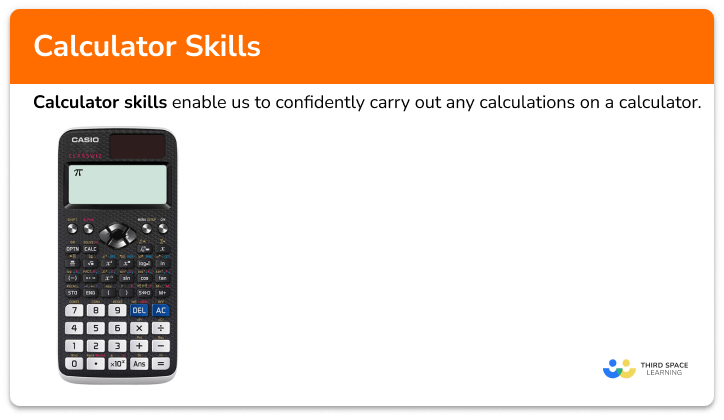Calculator skills

Step by step guide, detailed examples, practice questions, exam questions and calculator skills worksheets.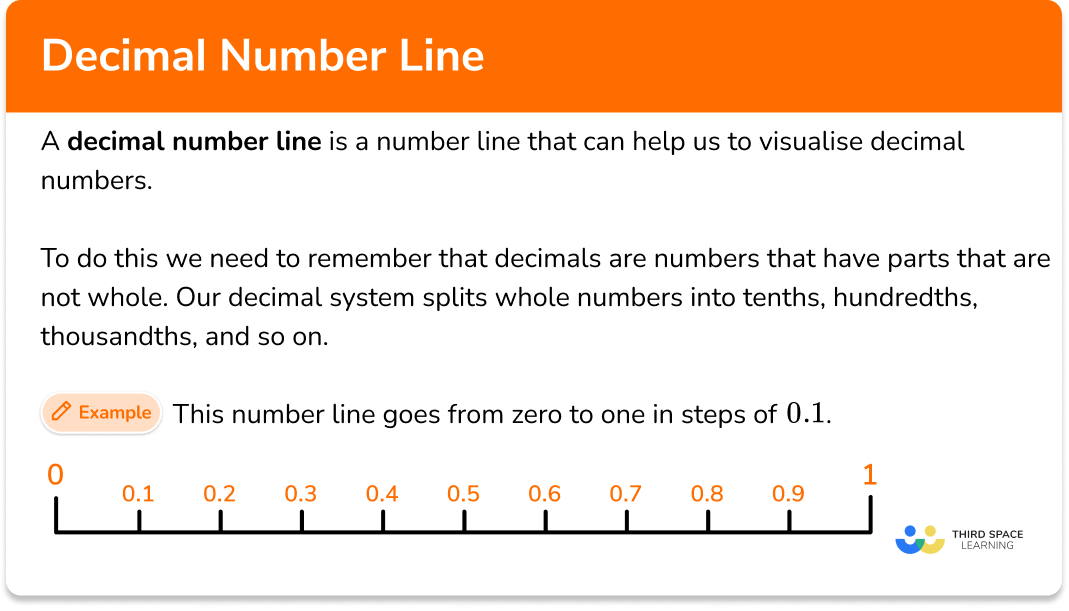Decimal number line

Step by step guide, detailed examples, practice questions, exam questions and decimal number line worksheets.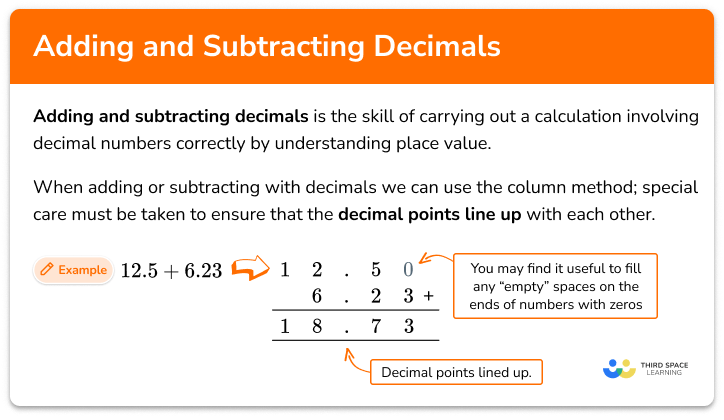Step by step guide, detailed examples, practice questions, exam questions and adding and subtracting decimals worksheets.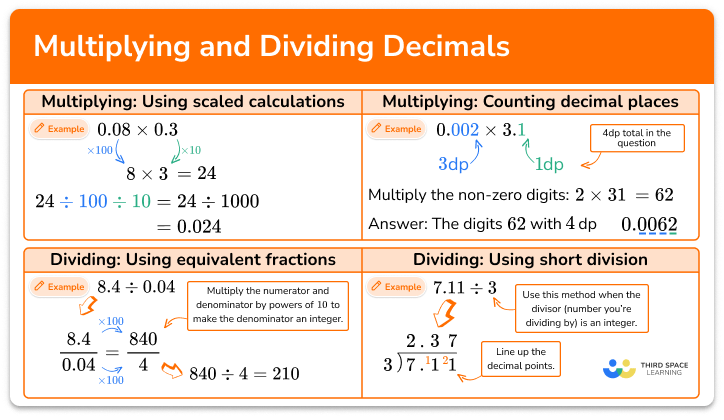Multiplying and dividing decimals

Step by step guide, detailed examples, practice questions, exam questions and multiplying and dividing decimals worksheets.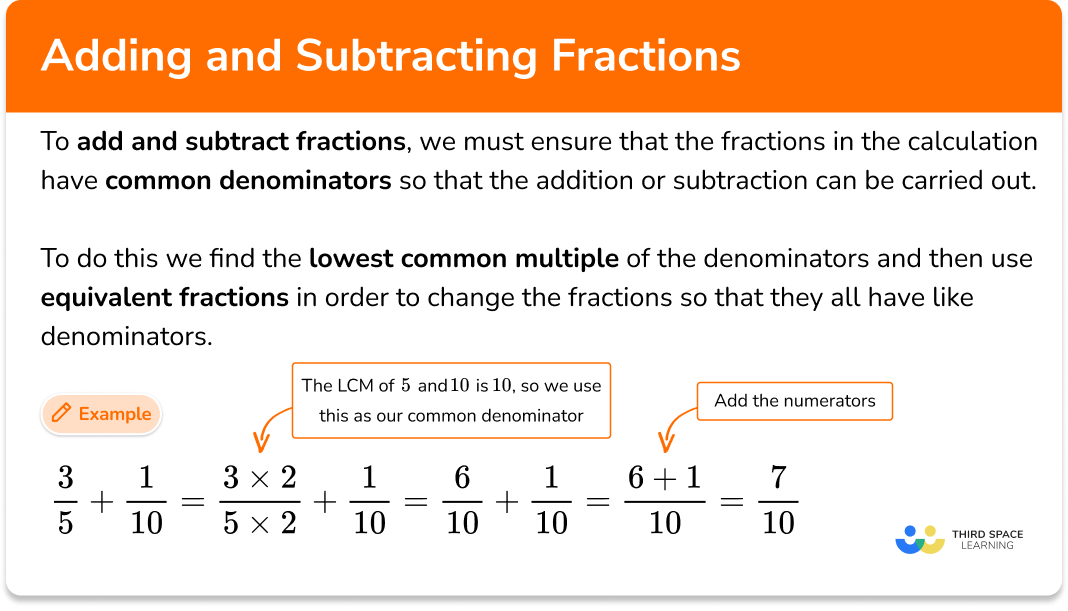Step by step guide, detailed examples, practice questions, exam questions and adding and subtracting fractions worksheets.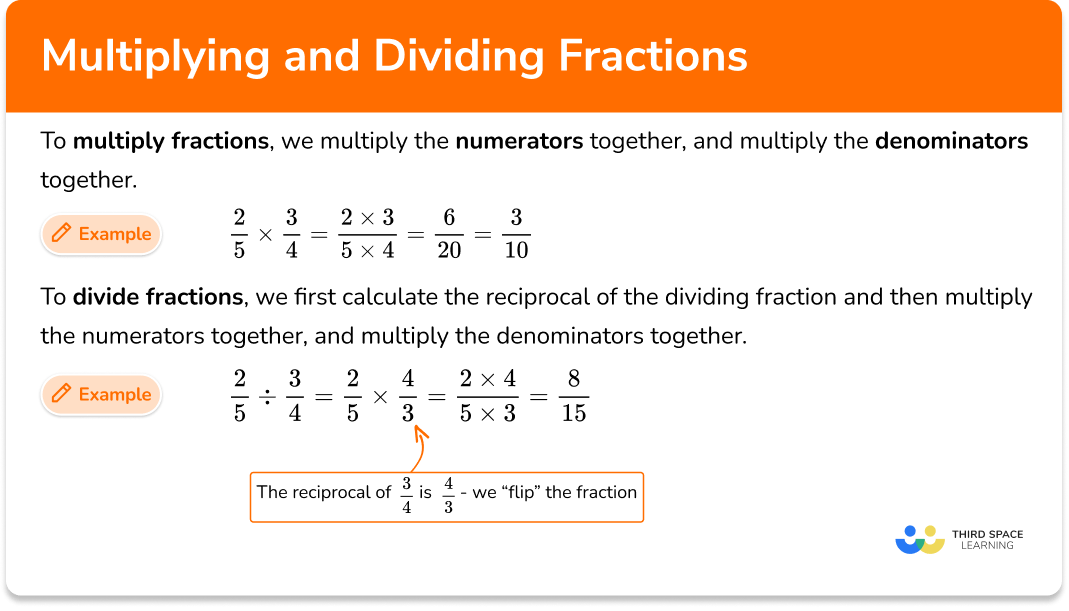Multiplying and dividing fractions

Step by step guide, detailed examples, practice questions, exam questions and multiplying and dividing fractions worksheets.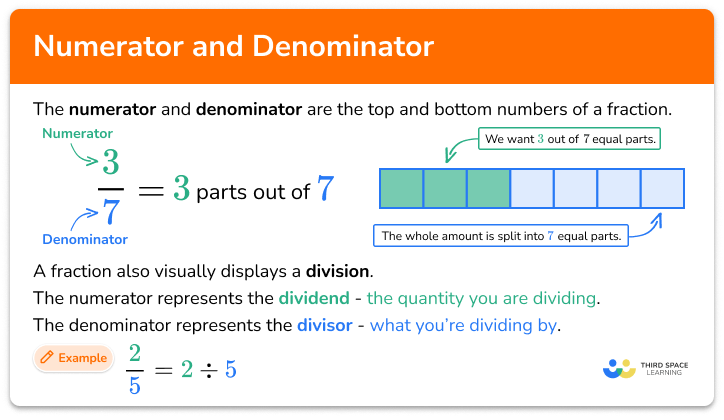Numerator and denominator

Step by step guide, detailed examples, practice questions, exam questions and numerator and denominator worksheets.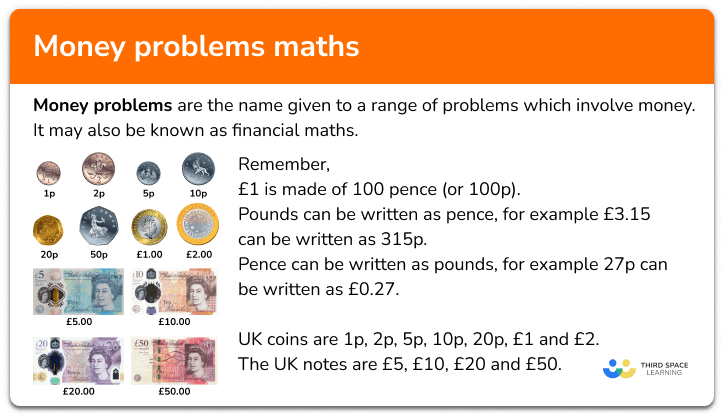Money problems maths

Step by step guide, detailed examples, practice questions, exam questions and money problems maths worksheets.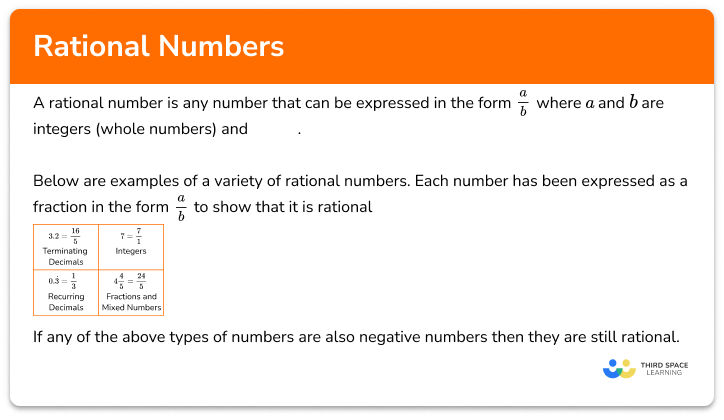Rational numbers

Step by step guide, detailed examples, practice questions, exam questions and rational numbers worksheets.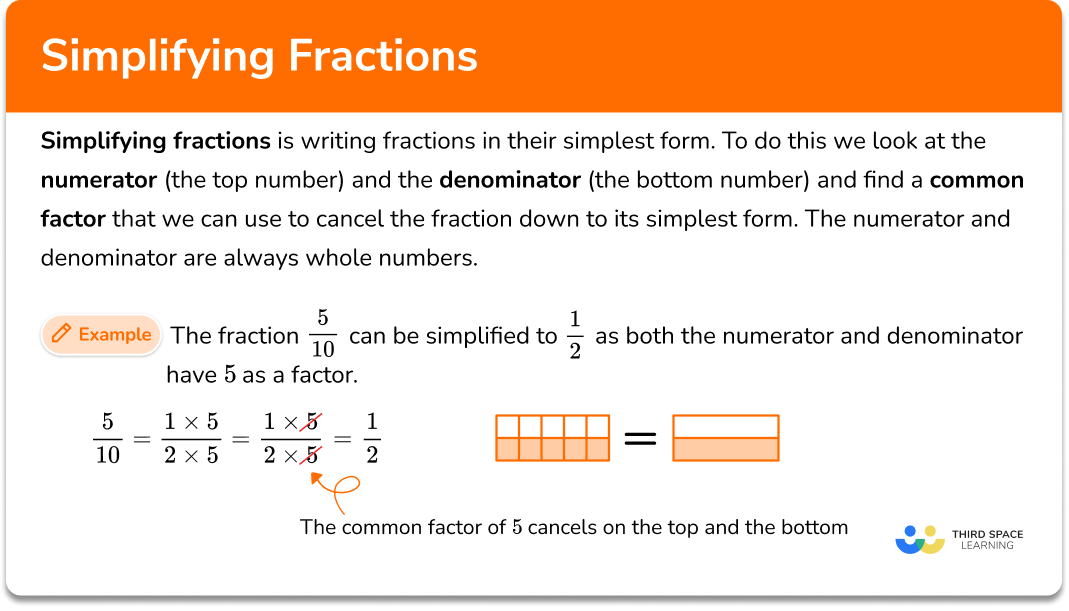Simplifying fractions

Step by step guide, detailed examples, practice questions, exam questions and simplifying fractions worksheets.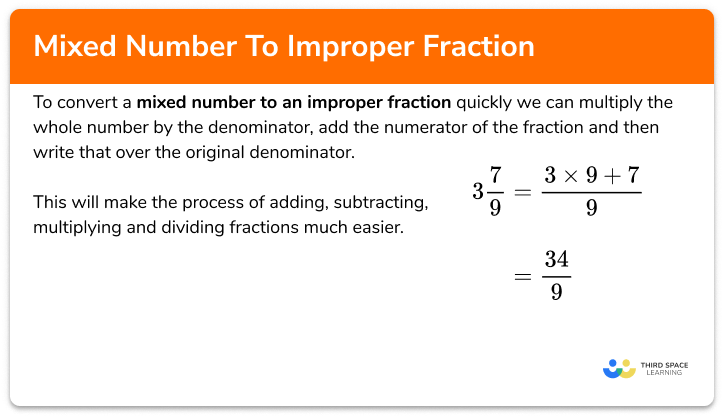Mixed number to improper fraction

Step by step guide, detailed examples, practice questions, exam questions and mixed number to improper fraction worksheets.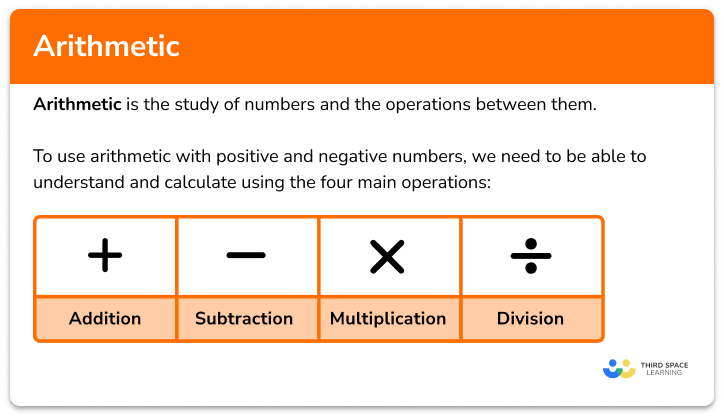Arithmetic

Step by step guide, detailed examples, practice questions, exam questions and arithmetic worksheets.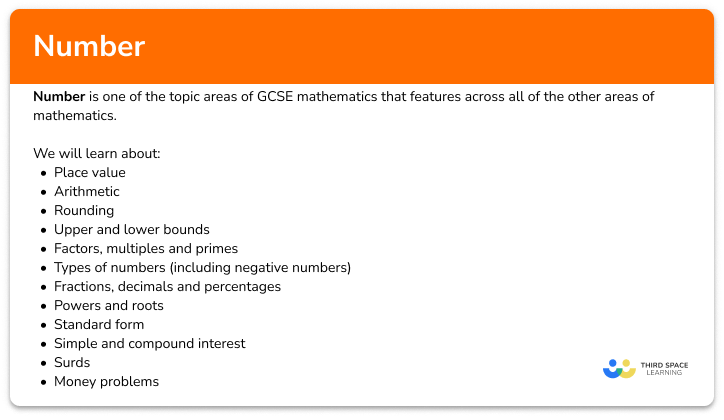Number – Maths GCSE

Step by step guide, detailed examples, practice questions, exam questions and gcse number worksheets.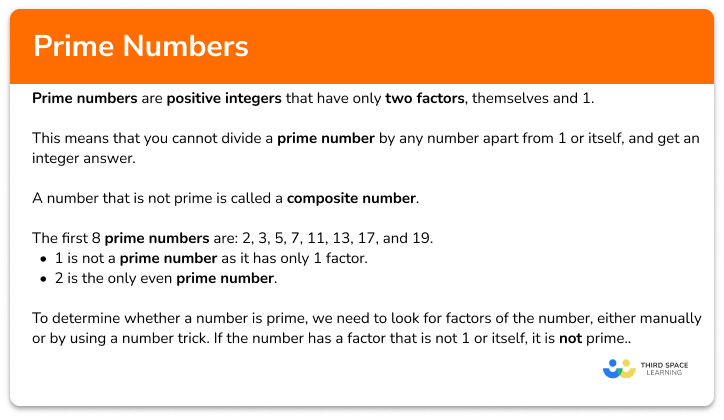Prime numbers

Step by step guide, detailed examples, practice questions, exam questions and prime numbers worksheets.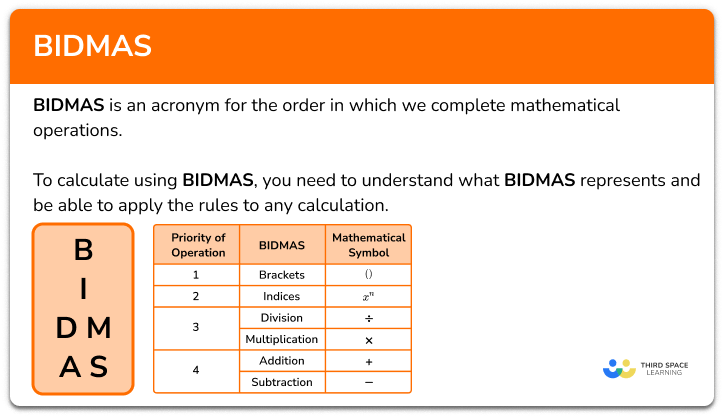BIDMAS

Step by step guide, detailed examples, practice questions, exam questions and bidmas worksheets.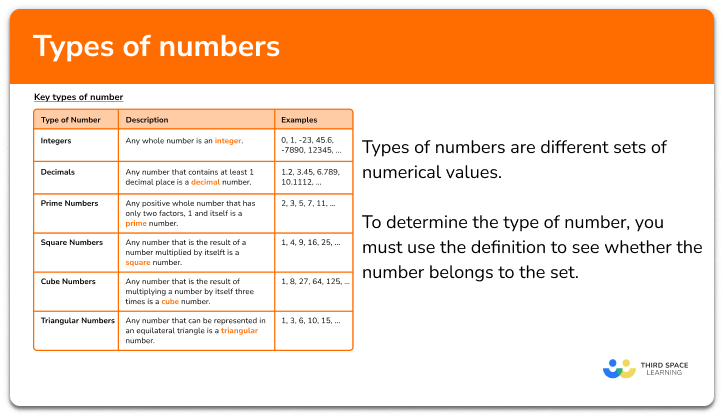Types of numbers

Step by step guide, detailed examples, practice questions, exam questions and types of numbers worksheets.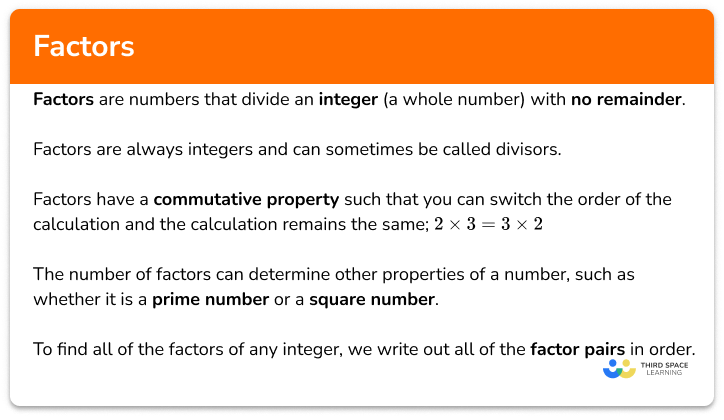Factors

Step by step guide, detailed examples, practice questions, exam questions and factors worksheets.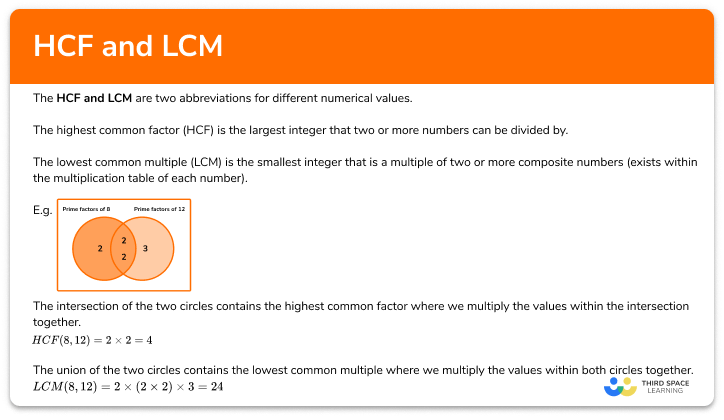HCF and LCM

Step by step guide, detailed examples, practice questions, exam questions and hcf and lcm worksheets.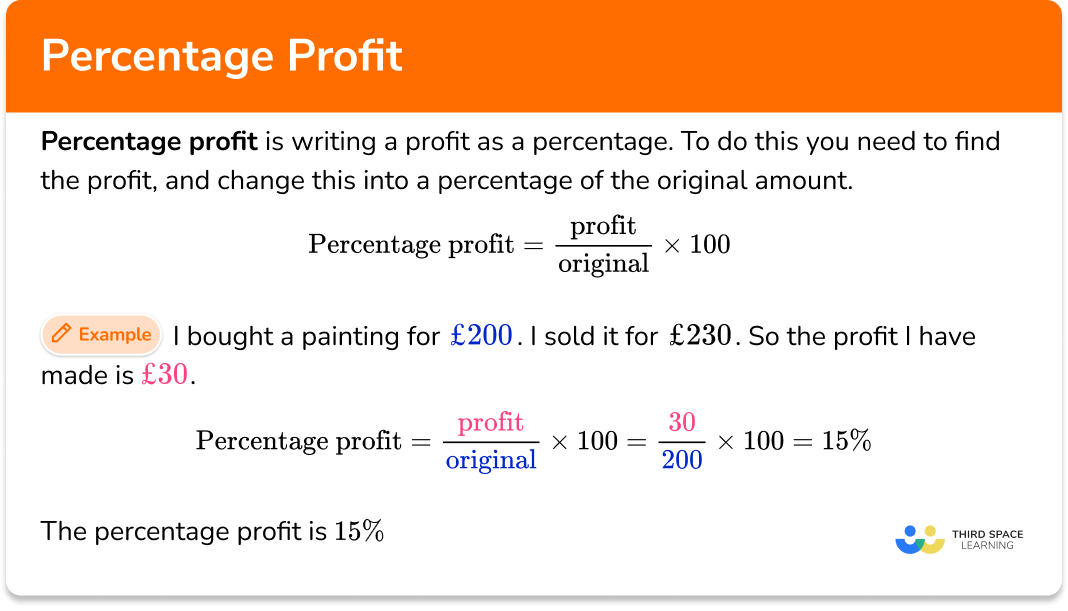Percentage profit

Step by step guide, detailed examples, practice questions, exam questions and percentage profit worksheets.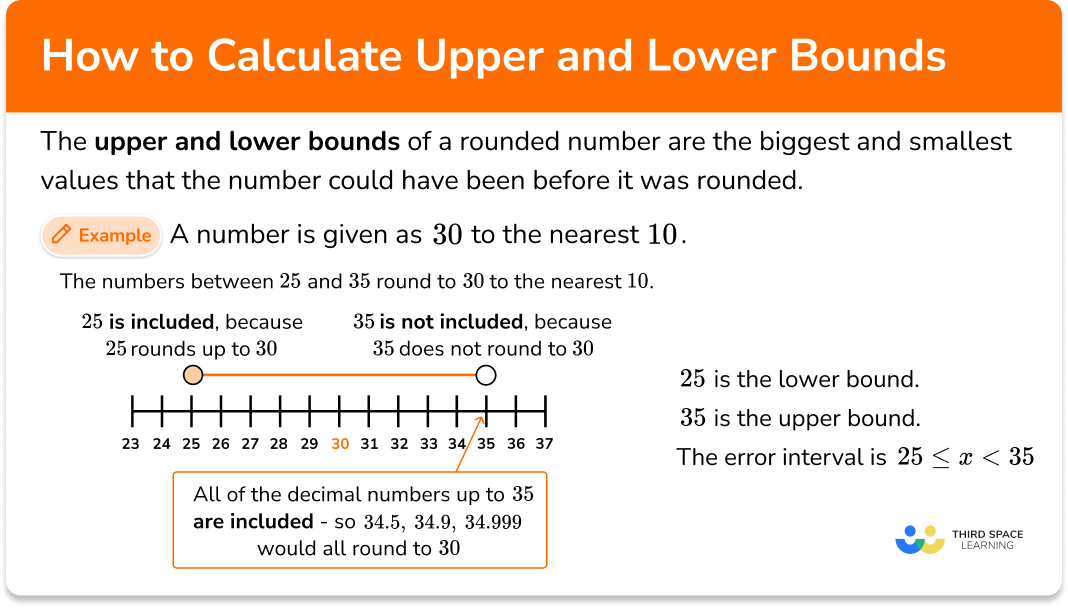How to calculate upper and lower bounds

Step by step guide, detailed examples, practice questions, exam questions and how to calculate upper and lower bounds worksheets.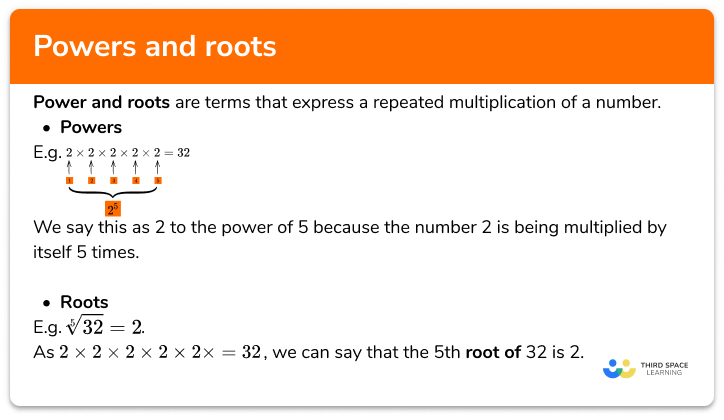Powers and roots

Step by step guide, detailed examples, practice questions, exam questions and powers and roots worksheets.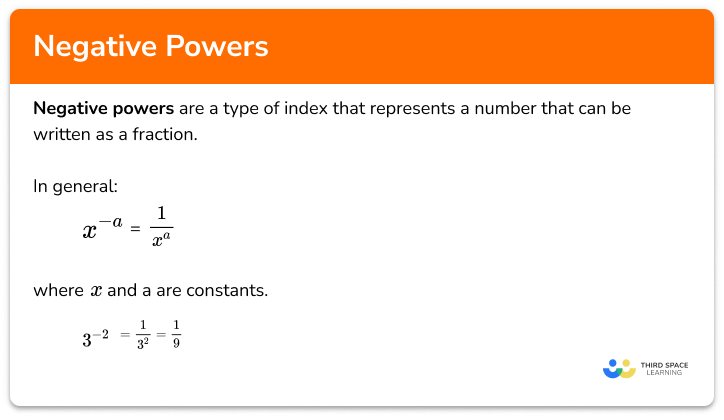Negative powers

Step by step guide, detailed examples, practice questions, exam questions and negative powers worksheets.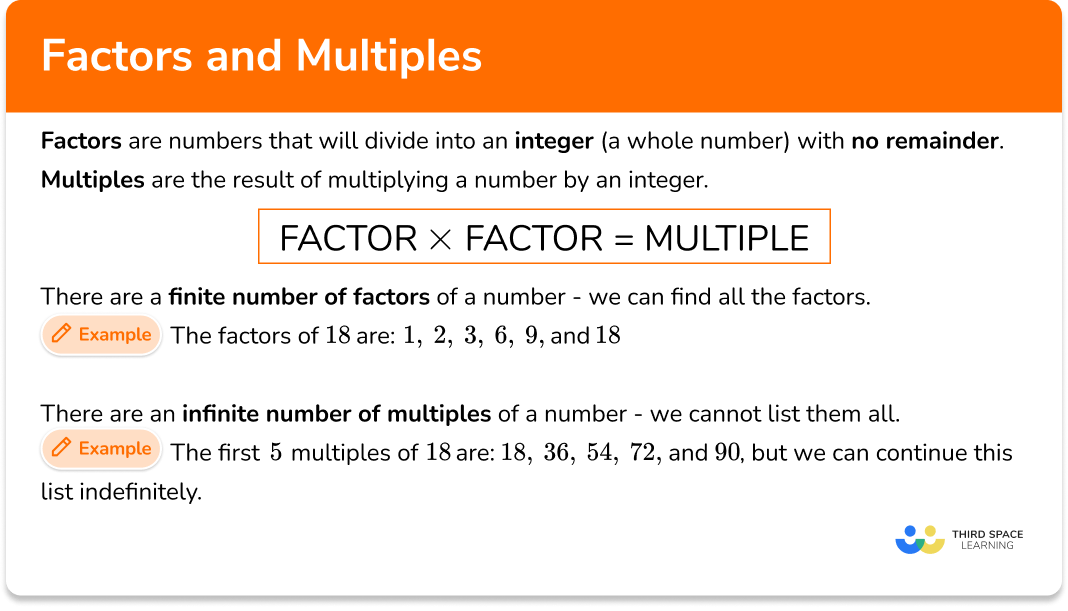Factors and multiples

Step by step guide, detailed examples, practice questions, exam questions and factors and multiples worksheets.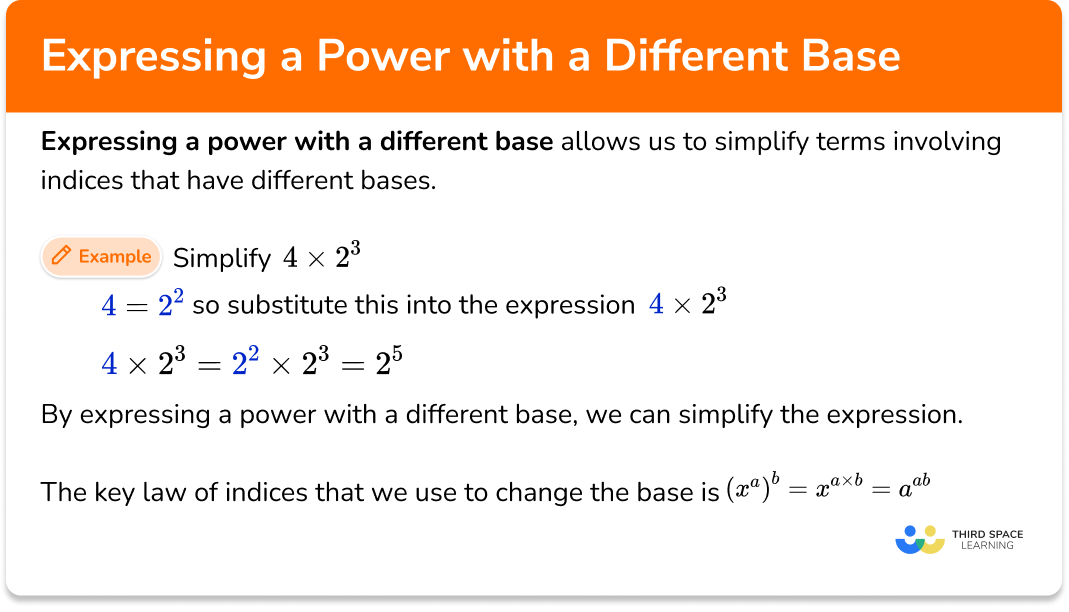Expressing a power with a different base

Step by step guide, detailed examples, practice questions, exam questions and expressing a power with a different base worksheets.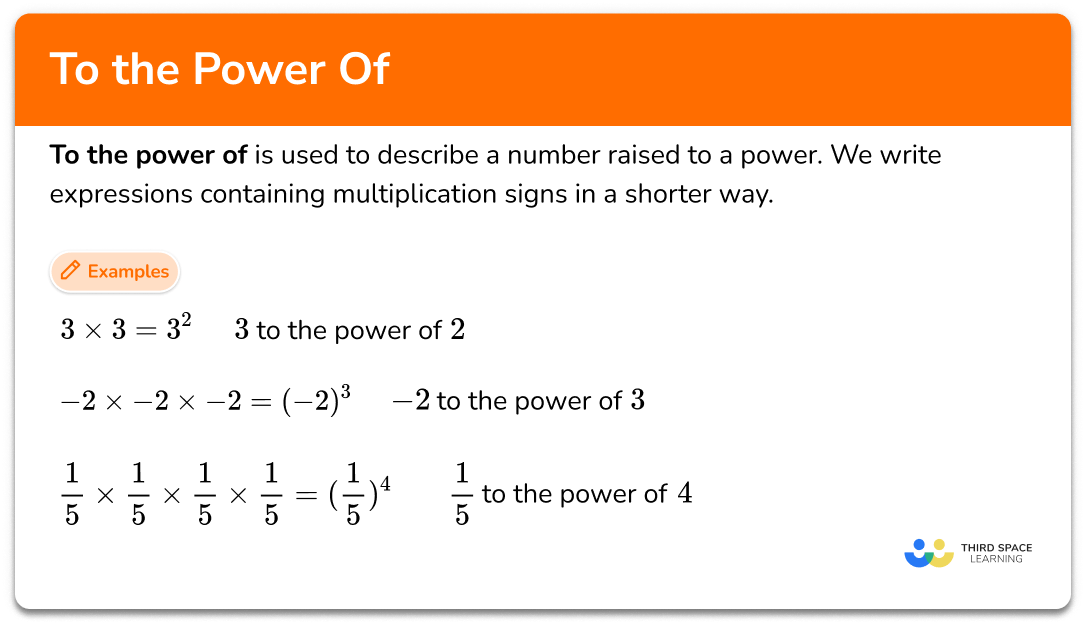To the power of

Step by step guide, detailed examples, practice questions, exam questions and to the power of worksheets.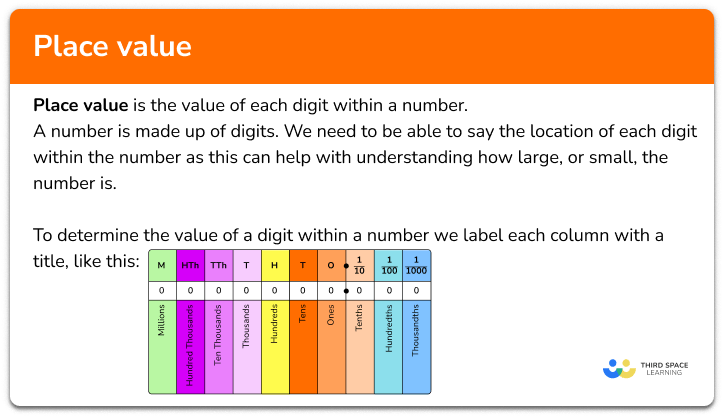Place value

Step by step guide, detailed examples, practice questions, exam questions and place value worksheets.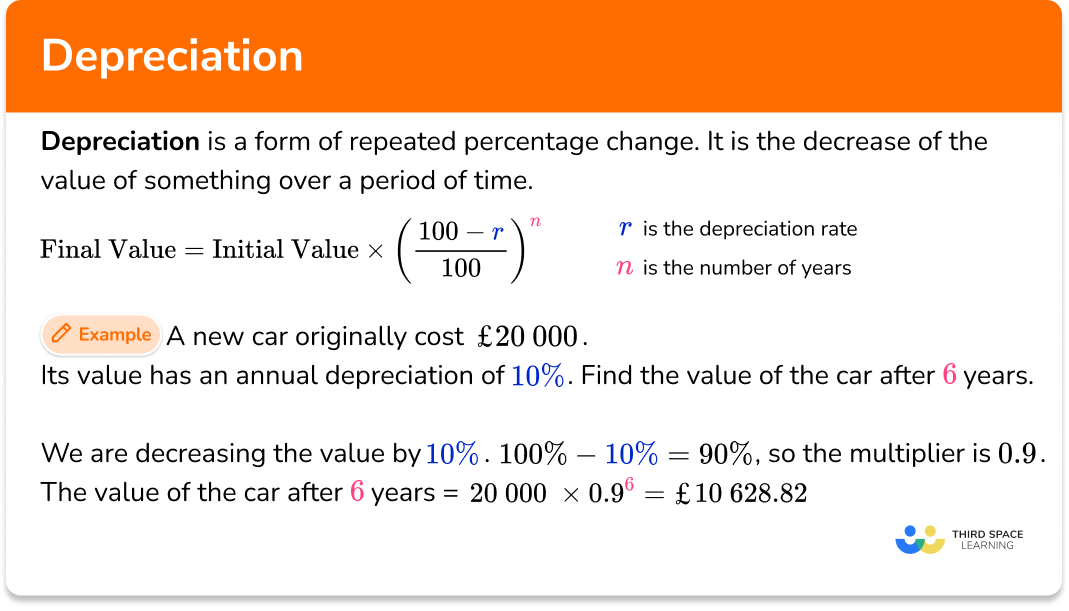Depreciation

Step by step guide, detailed examples, practice questions, exam questions and depreciation worksheets.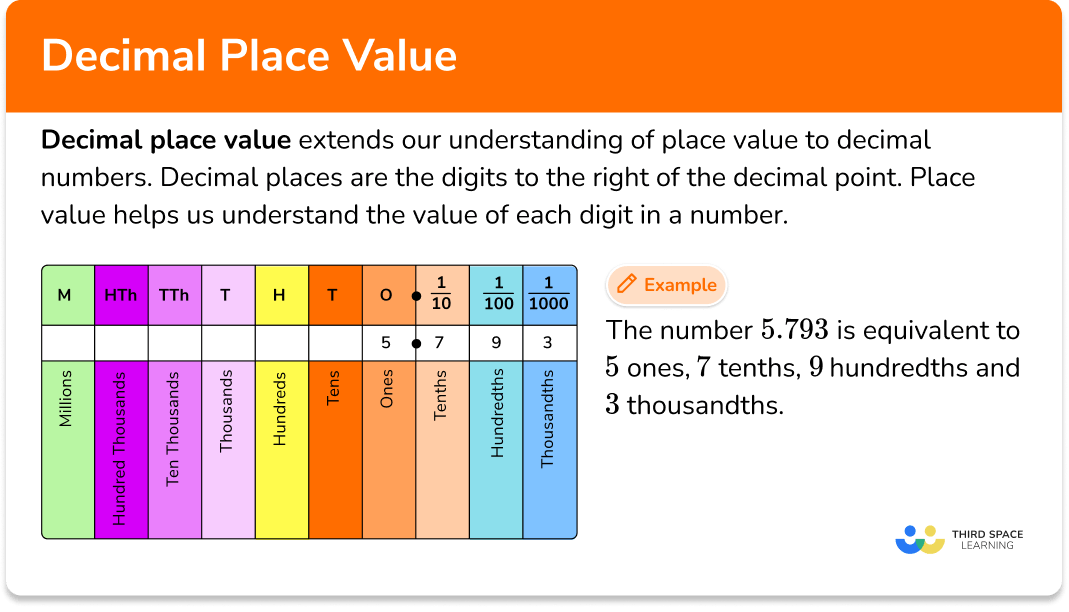Decimal place value

Step by step guide, detailed examples, practice questions, exam questions and decimal place value worksheets.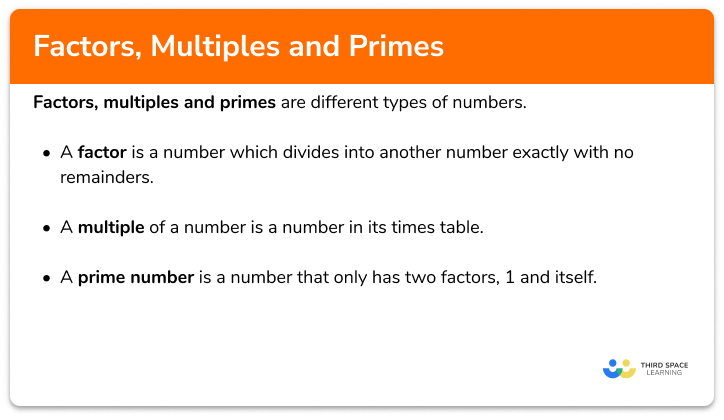Factors, multiples and primes

Step by step guide, detailed examples, practice questions, exam questions and factors and multiples worksheets.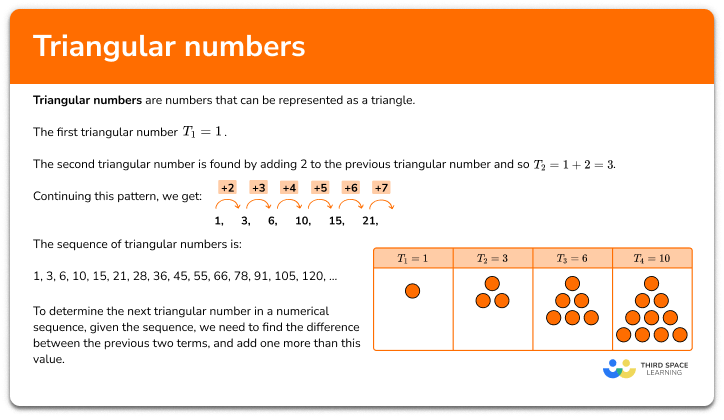Triangular numbers

Step by step guide, detailed examples, practice questions, exam questions and triangular numbers worksheets.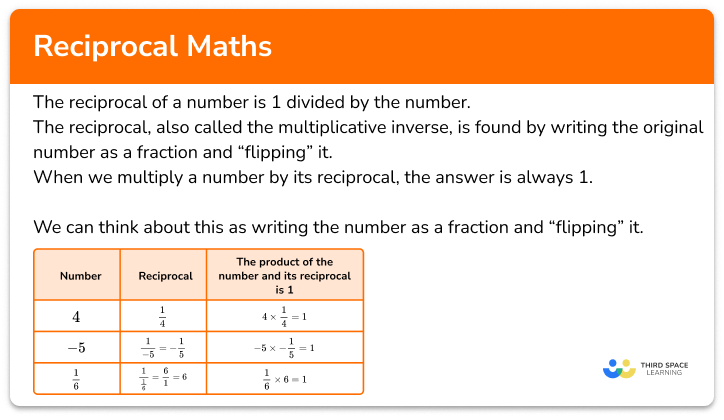Reciprocal maths

Step by step guide, detailed examples, practice questions, exam questions and reciprocal maths worksheets.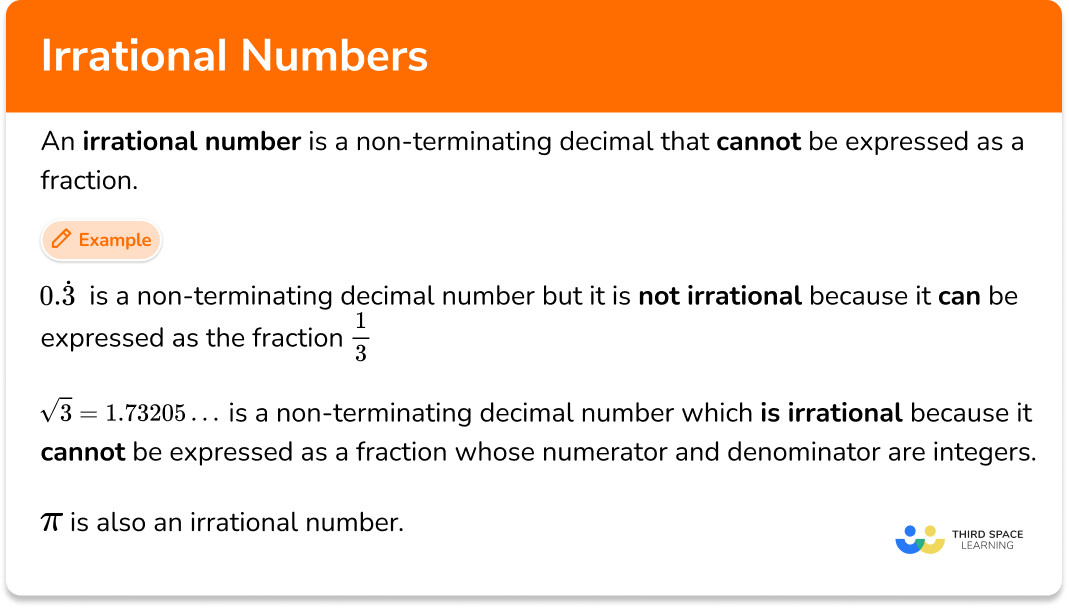Irrational numbers

Step by step guide, detailed examples, practice questions, exam questions and irrational numbers worksheets.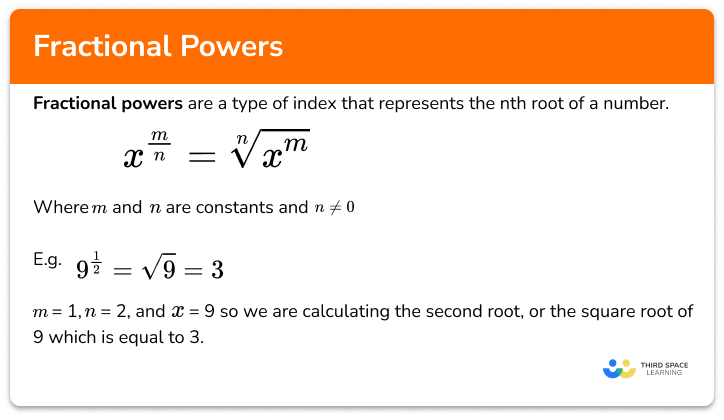Fractional powers

Step by step guide, detailed examples, practice questions, exam questions and fractional powers worksheets.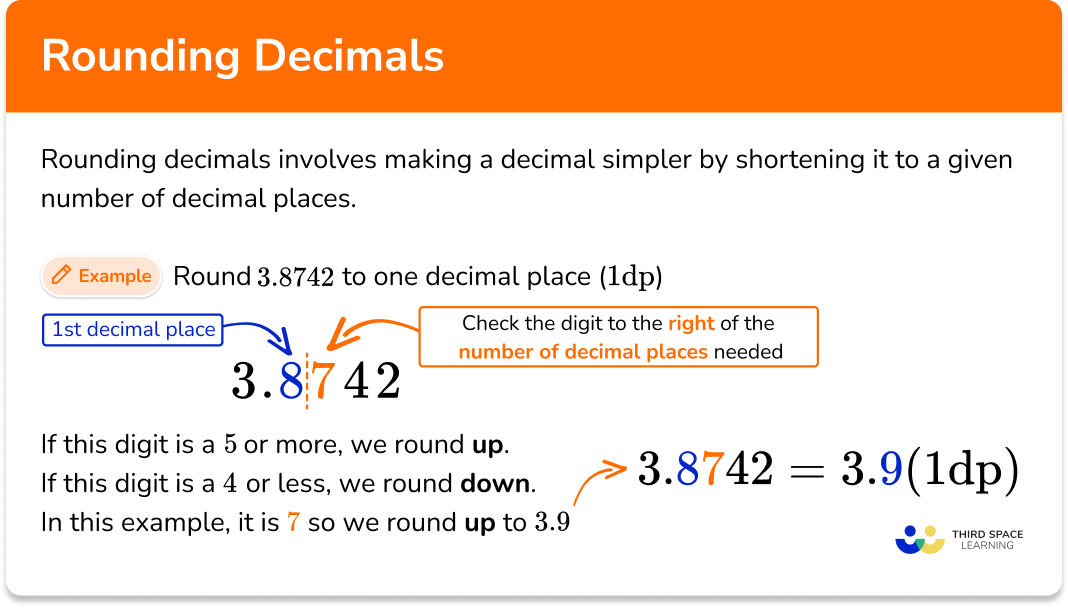Rounding decimals

Step by step guide, detailed examples, practice questions, exam questions and rounding decimals worksheets.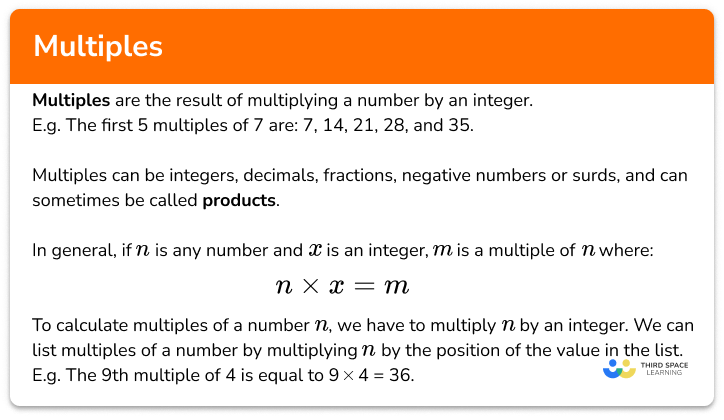Multiples

Step by step guide, detailed examples, practice questions, exam questions and multiples worksheets.

One to one maths interventions built for KS4 success

Weekly online one to one GCSE maths revision lessons now available